• 机器学习算法简介1.1 按照学习方式分类1.2 区别1.3 关于监督学习中分类与回归区别1.4 机器学习开发流程2. 数据集介绍与划分2.1 sklearn数据集介绍2.1.1 分类回归数据集2.1.2 API2.1.3 返回类型2.1.4 代码分析...
机器学习分类算法1. 机器学习算法简介1.1 按照学习方式分类1.2 区别1.3 关于监督学习中的分类与回归区别1.4 机器学习开发流程2. 数据集介绍与划分2.1 sklearn数据集介绍2.1.1 分类和回归数据集2.1.2 API2.1.3 返回类型2.1.4 代码分析2.2 转换器和估计器2.2.1转换器2.2.2 估计器(sklearn机器学习算法的实现)2.2.3 估计器工作流程3. K-近邻算法3.1K-近邻算法(KNN)3.1.1 定义3.1.2 距离公式3.2 电影类型分析3.3 K-近邻算法API3.4 案例：预测签到位置3.4.1 分析3.4.2 代码3.4.3 结果分析3.5 总结4 模型选择与调优4.1 为什么需要交叉验证4.2 什么是交叉验证(cross validation)4.3 超参数搜索-网格搜索(Grid Search)4.4 Facebook签到位置预测K值调优5.朴素贝叶斯算法5.1 什么是朴素贝叶斯分类方法5.2 概率基础5.2.1 概率(Probability)定义5.2.2 女神是否喜欢计算案例5.2.3 条件概率与联合概率5.3 贝叶斯公式5.3.1 公式5.3.2 文章分类计算5.3.3 拉普拉斯平滑系数5.3.4 API5.4 案例：20类新闻分类5.4.1 分析5.4.2 代码5.5 总结6.决策树6.1 认识决策树6.2 决策树分类原理详解6.2.1 原理6.2.2 信息熵6.2.2.1 信息熵的定义6.2.2.2 总结（重要）6.2.3 决策树的划分依据之一------信息增益6.2.3.1 定义与公式6.2.3.2 贷款特征重要计算6.2.4 决策树的三种算法实现6.2.5 决策树API6.3 案例：泰坦尼克号乘客生存预测6.3.1 分析6.3.2 保存树的结构到dot文件6.3.3 代码6.4 总结7. 集成学习方法之随机森林7.1 什么是集成学习方法7.2 什么是随机森林7.3 随机森林原理过程7.3.1 为什么采用BootStrap抽样7.3.2 API7.3.3 代码7.4 总结
1. 机器学习算法简介
学习目标:
说明机器学习算法监督学习与无监督学习的区别
说明机器学习算法目标值的两种数据类型
说明监督学习中的分类、回归特点
说明机器学习(数据挖掘)的开发流程
知道数据集的分为训练集和测试集
知道sklearn的转换器和估计器流程
了解sklearn的分类、回归数据集
说明K-近邻算法的距离公式
说明K-近邻算法的超参数K值以及取值问题
说明K-近邻算法的优缺点
应用KNeighborsClassifier实现分类
了解分类算法的评估标准准确率
说明朴素贝叶斯算法的原理
说明朴素贝叶斯算法的优缺点
应用MultinomialNB实现文本分类
应用模型选择与调优
说明决策树算法的原理
说明决策树算法的优缺点
应用DecisionTreeClassifier实现分类
说明随机森林算法的原理
说明随机森林算法的优缺点
应用RandomForestClassifier实现分类
1.1 按照学习方式分类
监督学习(supervised learning)（预测）
定义：输入数据是由输入特征值和目标值所组成。函数的输出可以是一个连续的值(称为回归），或是输出是有限个离散值（称作分类）。
分类: k-近邻算法、贝叶斯分类、决策树与随机森林、逻辑回归、神经网络
回归: 线性回归、岭回归
标注 隐马尔可夫模型 (不做要求)
无监督学习(unsupervised learning)
定义：输入数据是由输入特征值所组成。
聚类 k-means
半监督和强化学习
1.2 区别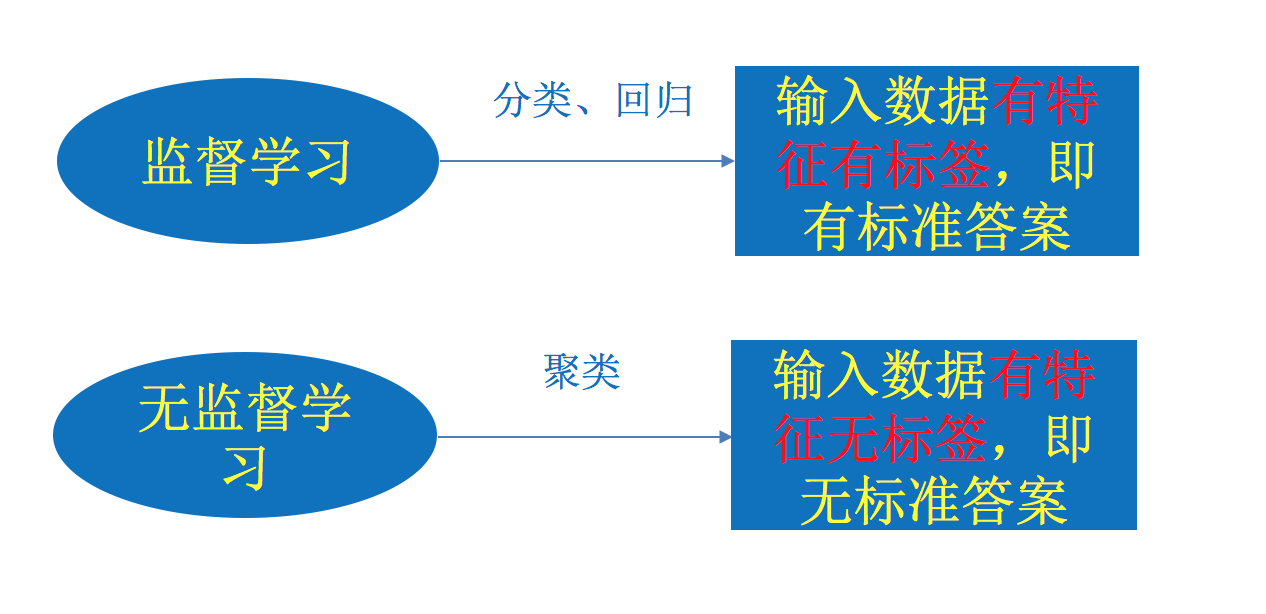1.3 关于监督学习中的分类与回归区别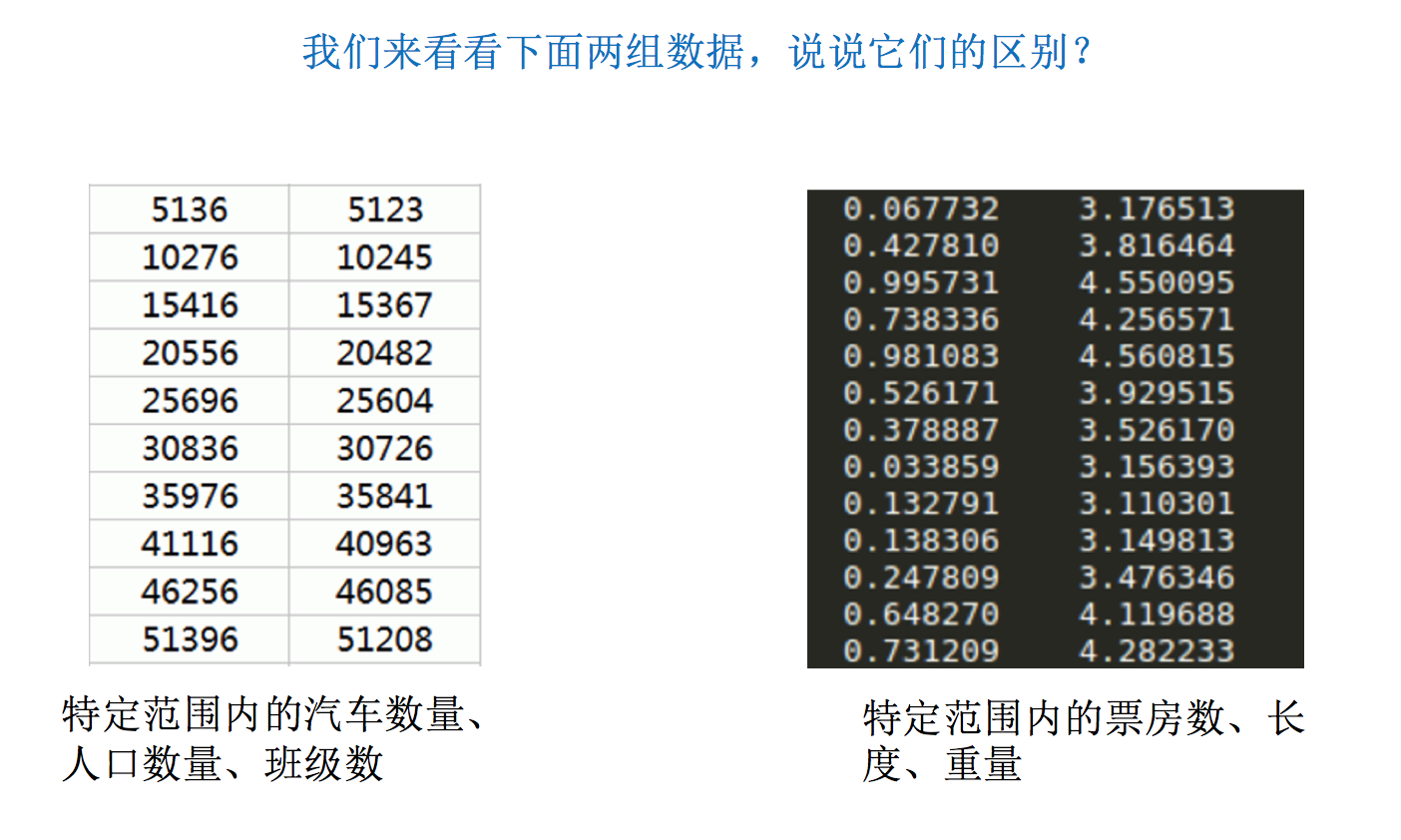两种数据类型:
离散型数据：由记录不同类别个体的数目所得到的数据，又称计数数据，所有这些数据全部都是整数，而且不能再细分，也不能进一步提高他们的精确度。
连续型数据：变量可以在某个范围内取任一数，即变量的取值可以是连续的，如，长度、时间、质量值等，这类整数通常是非整数，含有小数部分。
注：只要记住一点，离散型是区间内不可分，连续型是区间内可分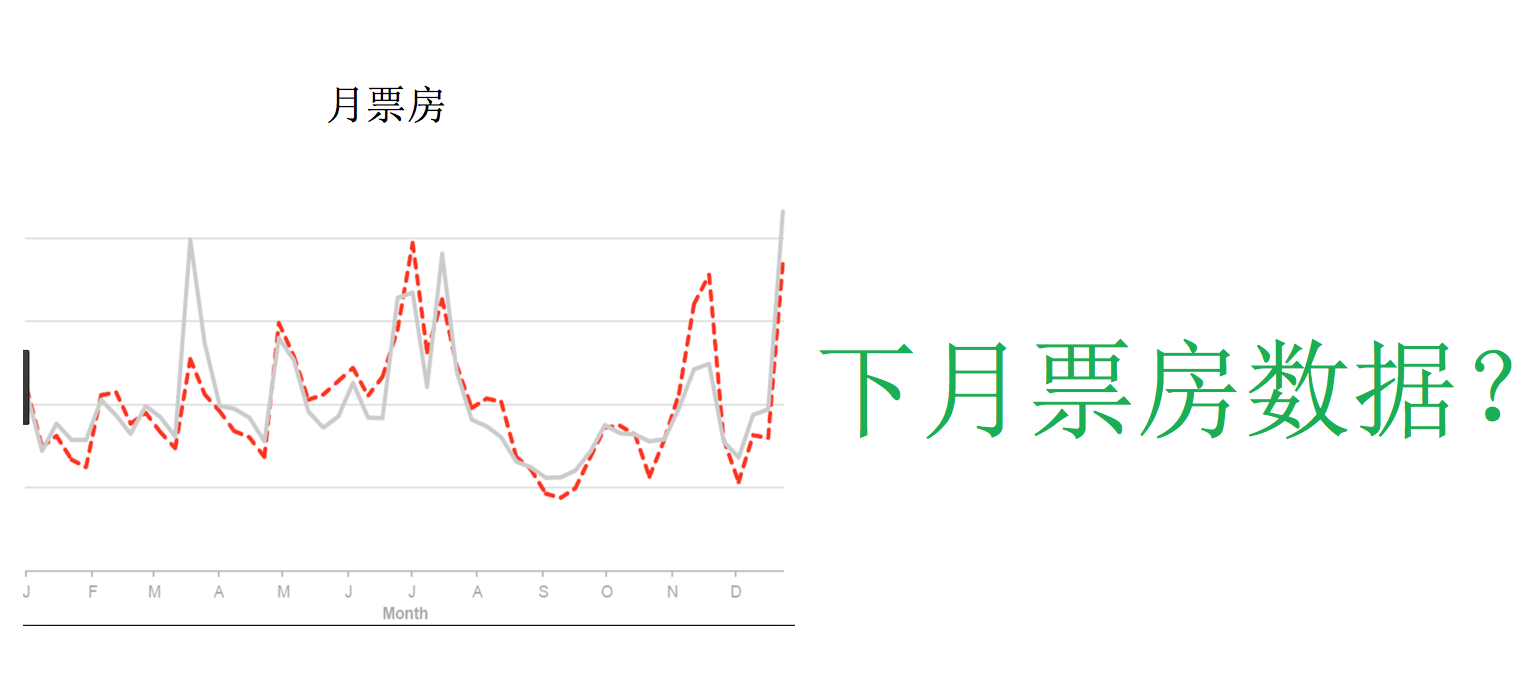结合刚才讲过的数据类型，针对这两个例子的输出结果，得出结论
分类：目标值数据类型为离散型
分类是监督学习的一个核心问题，在监督学习中，当输出变量取有限个离散值时，预测问题变成为分类问题，最基础的便是二分类问题，即判断是非，从两个类别中选择一个作为预测结果；
回归：目标值数据类型为连续型
回归是监督学习的另一个重要问题。回归用于预测输入变量和输出变量之间的关系，输出是连续型的值。
1.4 机器学习开发流程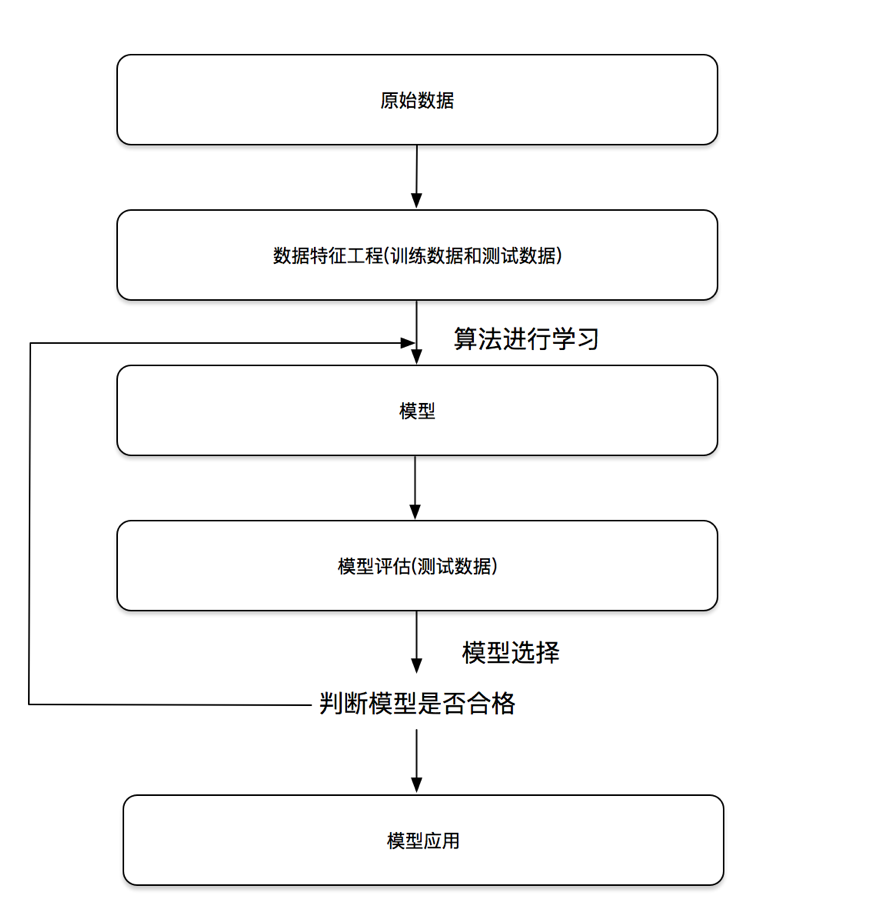2. 数据集介绍与划分
学习目标:
知道数据集的分为训练集和测试集
知道sklearn的分类、回归数据集
划分比例：
训练集：70% 80% 75%
测试集：30% 20% 30%
API:
sklearn.model_selection.train_test_split(arrays, *options)
x 数据集的特征值
y 数据集的标签值
test_size 测试集的大小，一般为float
random_state 随机数种子,不同的种子会造成不同的随机采样结果。相同的种子采样结果相同。
return ，测试集特征训练集特征值值，训练标签，测试标签(默认随机取)
结合后面的数据集作介绍
2.1 sklearn数据集介绍
2.1.1 分类和回归数据集
(1)分类数据集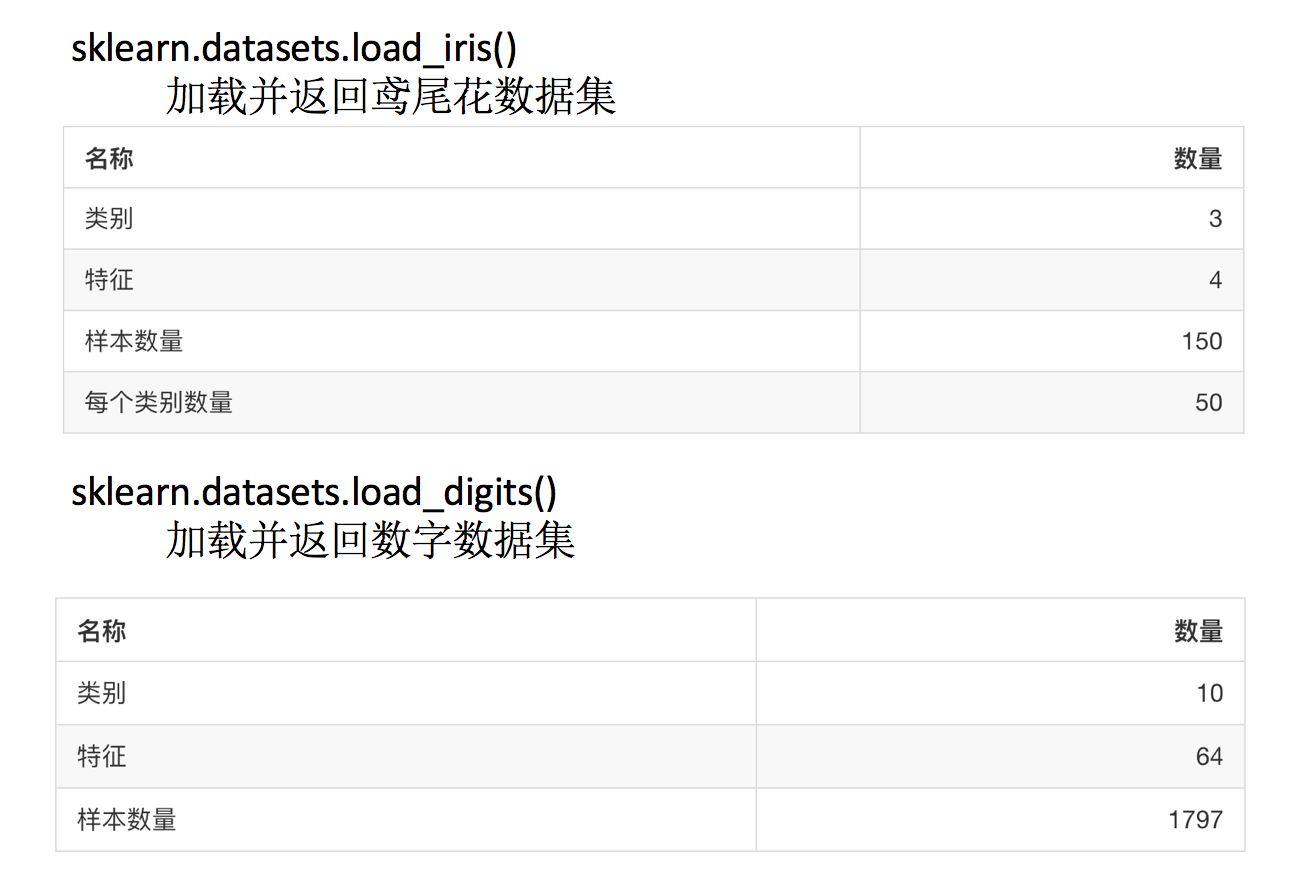sklearn.datasets.fetch_20newsgroups(data_home=None,subset=‘train’)
subset: ‘train’或者’test’,‘all’，可选，选择要加载的数据集.训练集的“训练”，测试集的“测试”，两者的“全部”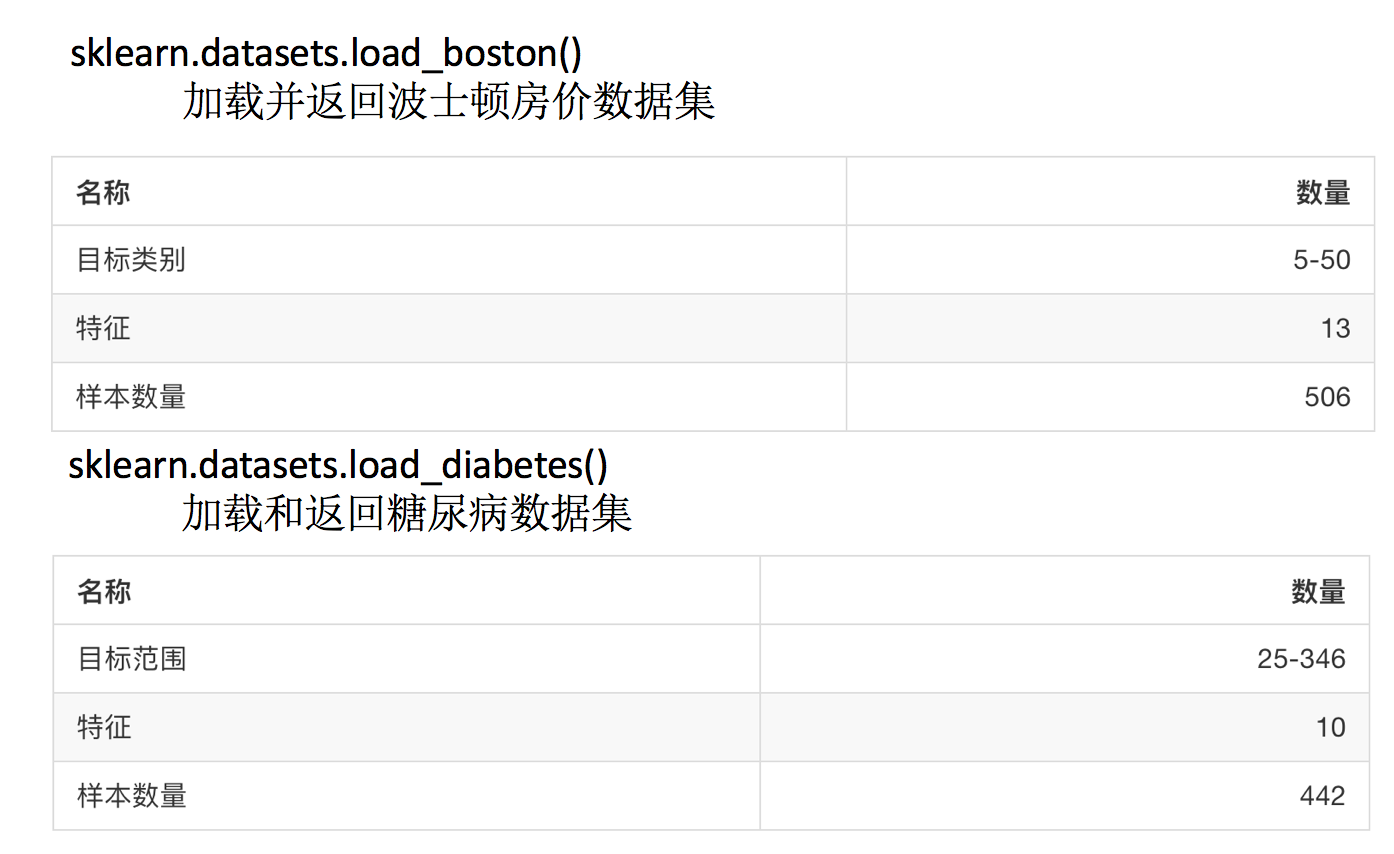2.1.2 API
sklearn.datasets     加载获取流行数据集
datasets.load_()
获取小规模数据集，数据包含在datasets里
datasets.fetch_(data_home=None)
获取大规模数据集，需要从网络上下载，函数的第一个参数是data_home，表示数据集下载的目录,默认是 ~/scikit_learn_data/
2.1.3 返回类型
load和fetch返回的数据类型datasets.base.Bunch(字典格式)
data：特征数据数组，是 [n_samples * n_features] 的二维 numpy.ndarray 数组
target：标签数组，是 n_samples 的一维     numpy.ndarray 数组
DESCR：数据描述
feature_names：特征名,新闻数据，手写数字、回归数据集没有
target_names：标签名
2.1.4 代码分析
from sklearn.datasets import load_iris, load_boston, fetch_20newsgroups
from sklearn.model_selection import train_test_split, GridSearchCV
#分类数据集
lr = load_iris()
# print("特征值", lr.data)
# print("目标值", lr.target)
# #print(lr.DESCR)
# print(lr.feature_names)
# print(lr.target_names)
#
# #回归数据集
# lb = load_boston()
# print("特征值：", lb.data)
# print("目标值:", lb.target)
#
# #数据量比较大的数据集
# news = fetch_20newsgroups(subset='all')
# print(news.data)
# print(news.target)
#
# #进行数据集的训练集和测试集划分
# #返回值有四个部分接收
# #x,y 特征值和目标值，train ,test训练集和测试集
# #x_train,x_test,y_train,y_test
x_train, x_test, y_train, y_test = train_test_split(lr.data, lr.target, test_size=0.3)

print("训练集的特征值：", x_train)
print("测试集的特征值", x_test)
print("训练集的目标值：", y_train)
print("测试集的目标值", y_test)

输出结果:
/home/yuyang/anaconda3/envs/tensor1-6/bin/python3.5 "/media/yuyang/Yinux/heima/Machine learning/demo.py"
训练集的特征值： [[5.2 3.4 1.4 0.2]
[6.3 2.8 5.1 1.5]
[6.9 3.1 4.9 1.5]
[5.8 2.7 3.9 1.2]
[5.2 4.1 1.5 0.1]
[5.9 3.2 4.8 1.8]
[5.8 2.8 5.1 2.4]
.......................
[4.9 3.1 1.5 0.1]
测试集的特征值 [[6.5 3.  5.2 2. ]
[5.7 4.4 1.5 0.4]
......................
[5.7 2.6 3.5 1. ]]
训练集的目标值： [0 2 1 1 0 1 2 0 2 2 2 1 2 1 2 0 1 2 2 2 1 0 1 1 1 2 2 1 0 2 1 2 2 1 0 2 0
1 1 2 0 0 1 0 0 1 2 0 0 2 0 0 1 0 1 0 0 0 0 1 1 0 1 2 1 2 1 2 2 2 2 0 2 2
1 1 0 0 2 0 0 1 0 1 2 0 0 2 2 0 1 2 0 1 1 1 2 2 0 2 0 2 2 2 0]
测试集的目标值 [2 0 1 1 1 1 2 0 0 0 2 2 0 0 0 1 2 2 0 0 2 1 1 1 1 0 0 1 2 1 1 1 0 0 2 2 2
1 2 1 0 0 1 1 1]

Process finished with exit code 0


2.2 转换器和估计器
2.2.1转换器
想一下之前做的特征工程的步骤？
1、实例化 (实例化的是一个转换器类(Transformer))
2、调用fit_transform(对于文档建立分类词频矩阵，不能同时调用)
我们把特征工程的接口称之为转换器，其中转换器调用有这么几种形式
fit_transform
fit
transform
这几个方法之间的区别是什么呢？我们看以下代码就清楚了
In : from sklearn.preprocessing import StandardScaler

In : std1 = StandardScaler()

In : a = [[1,2,3], [4,5,6]]

In : std1.fit_transform(a)
Out:
array([[-1., -1., -1.],
[ 1.,  1.,  1.]])

In : std2 = StandardScaler()

In : std2.fit(a)
Out: StandardScaler(copy=True, with_mean=True, with_std=True)

In : std2.transform(a)
Out:
array([[-1., -1., -1.],
[ 1.,  1.,  1.]])

从中可以看出，fit_transform的作用相当于transform加上fit。但是为什么还要提供单独的fit呢, 我们还是使用原来的std2来进行标准化看看
In : b = [[7,8,9], [10, 11, 12]]

In : std2.transform(b)
Out:
array([[3., 3., 3.],
[5., 5., 5.]])

In : std2.fit_transform(b)
Out:
array([[-1., -1., -1.],
[ 1.,  1.,  1.]])

注:此例说明
fit_transform=fit + transform
fit_transform(a):标准化:以自己a的平均值标准差转换自己
fit(a):计算a的平均值和标准差,transform(b):以a的平均值和标准差去转换b
适用于特征选择,主成分分析,特征抽取
2.2.2 估计器(sklearn机器学习算法的实现)
在sklearn中，估计器(estimator)是一个重要的角色，是一类实现了算法的API
1、用于分类的估计器：
sklearn.neighbors k-近邻算法
sklearn.naive_bayes 贝叶斯
sklearn.linear_model.LogisticRegression 逻辑回归
sklearn.tree 决策树与随机森林
2、用于回归的估计器：
sklearn.linear_model.LinearReg        ression 线性回归
sklearn.linear_model.Ridge 岭回归
3、用于无监督学习的估计器
2.2.3 估计器工作流程
sklearn.cluster.KMeans 聚类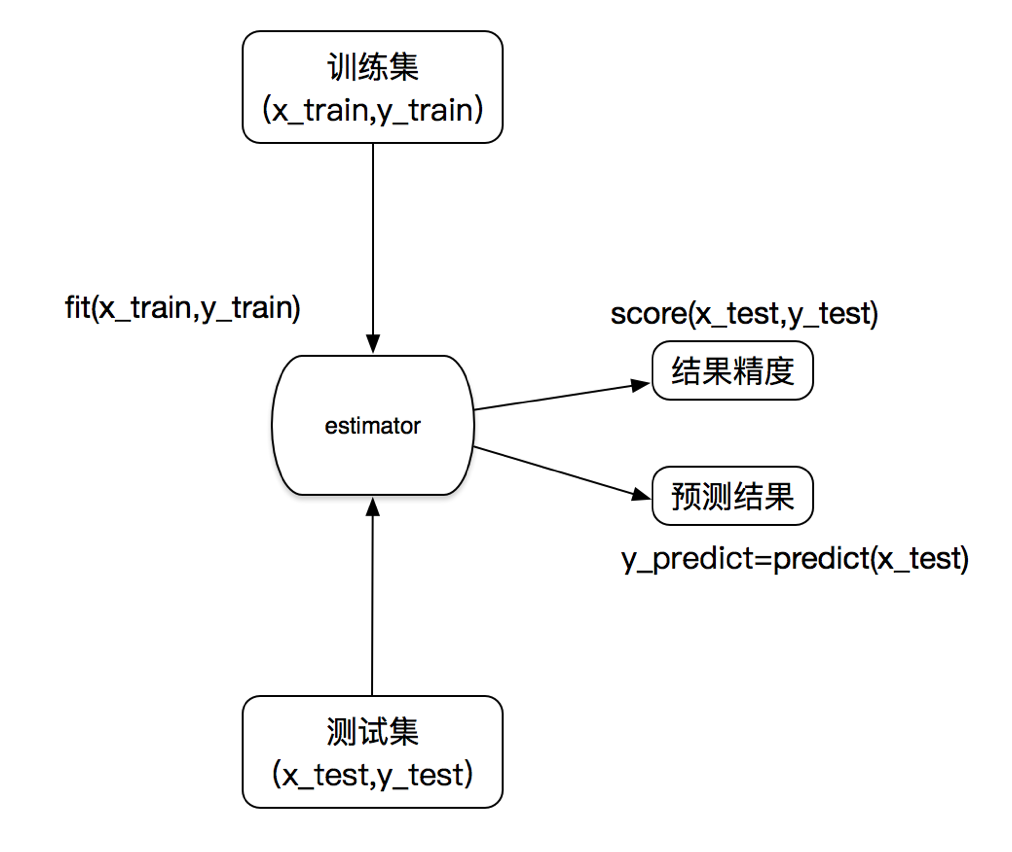estimator估计器:实现了大部分算法
fit():训练集训练
predict,score预测,准确率
3. K-近邻算法
学习目标:
说明K-近邻算法的距离公式
说明K-近邻算法的超参数K值以及取值问题
说明K-近邻算法的优缺点
应用KNeighborsClassifier实现分类
了解分类算法的评估标准准确率
应用
Facebook签到位置预测
什么是K-近邻算法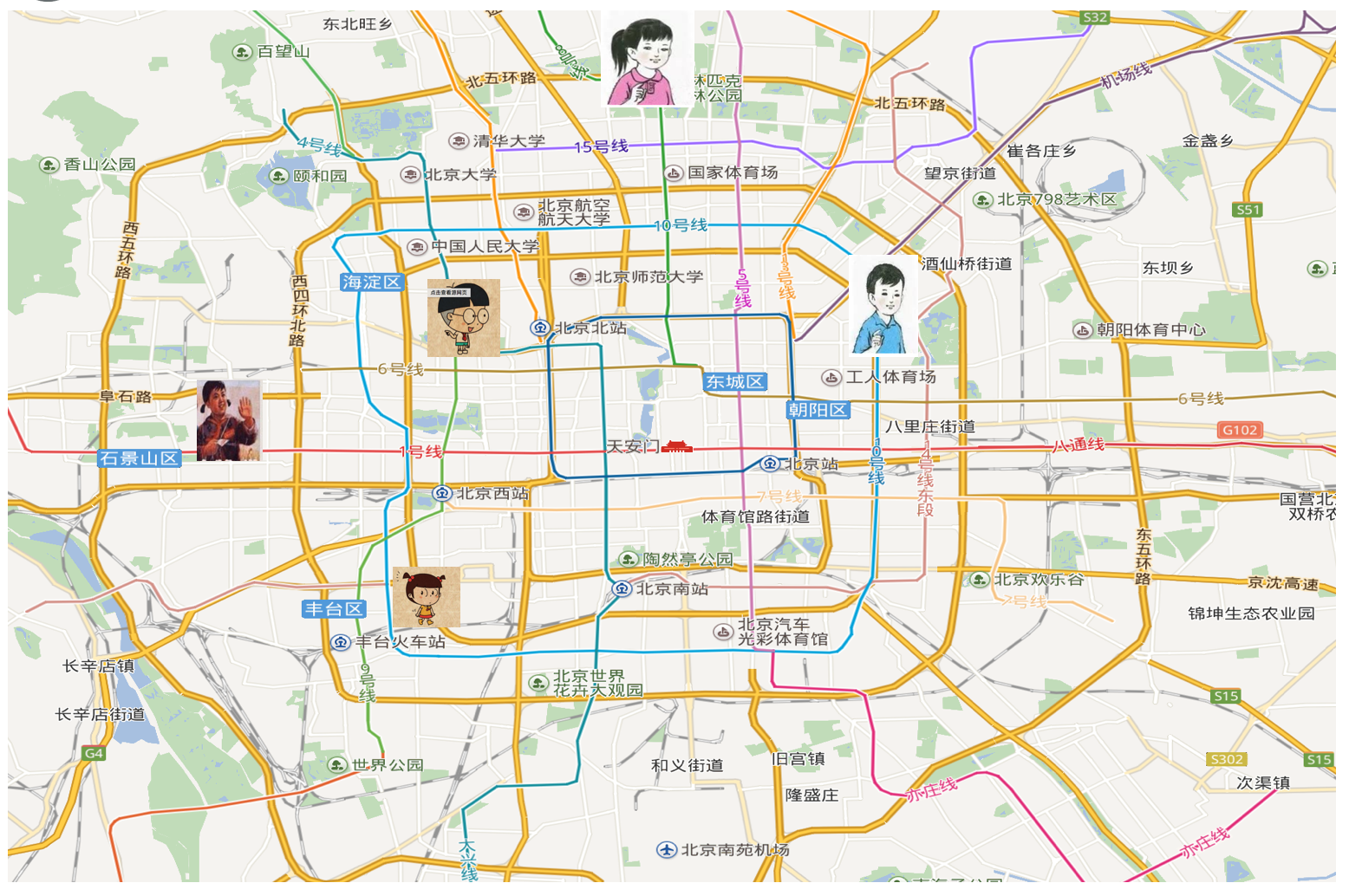你的“邻居”来推断出你的类别
3.1K-近邻算法(KNN)
3.1.1 定义
如果一个样本在特征空间中的k个最相似(即特征空间中最邻近)的样本中的大多数属于某一个类别，则该样本也属于这个类别。
来源：KNN算法最早是由Cover和Hart提出的一种分类算法

3.1.2 距离公式
两个样本的距离可以通过如下公式计算，又叫欧式距离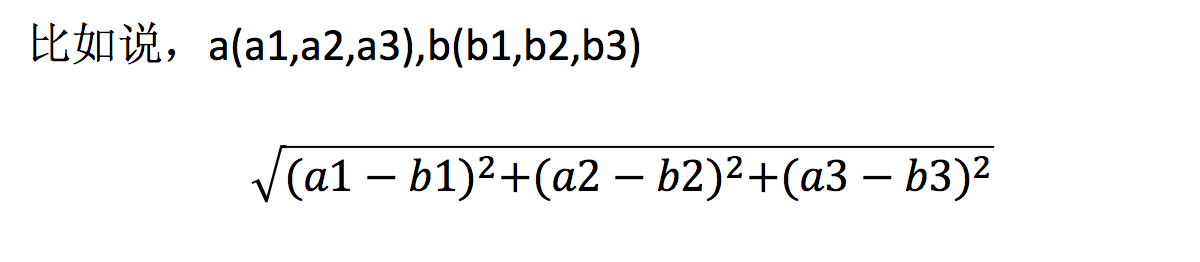3.2 电影类型分析
假设我们有现在几部电影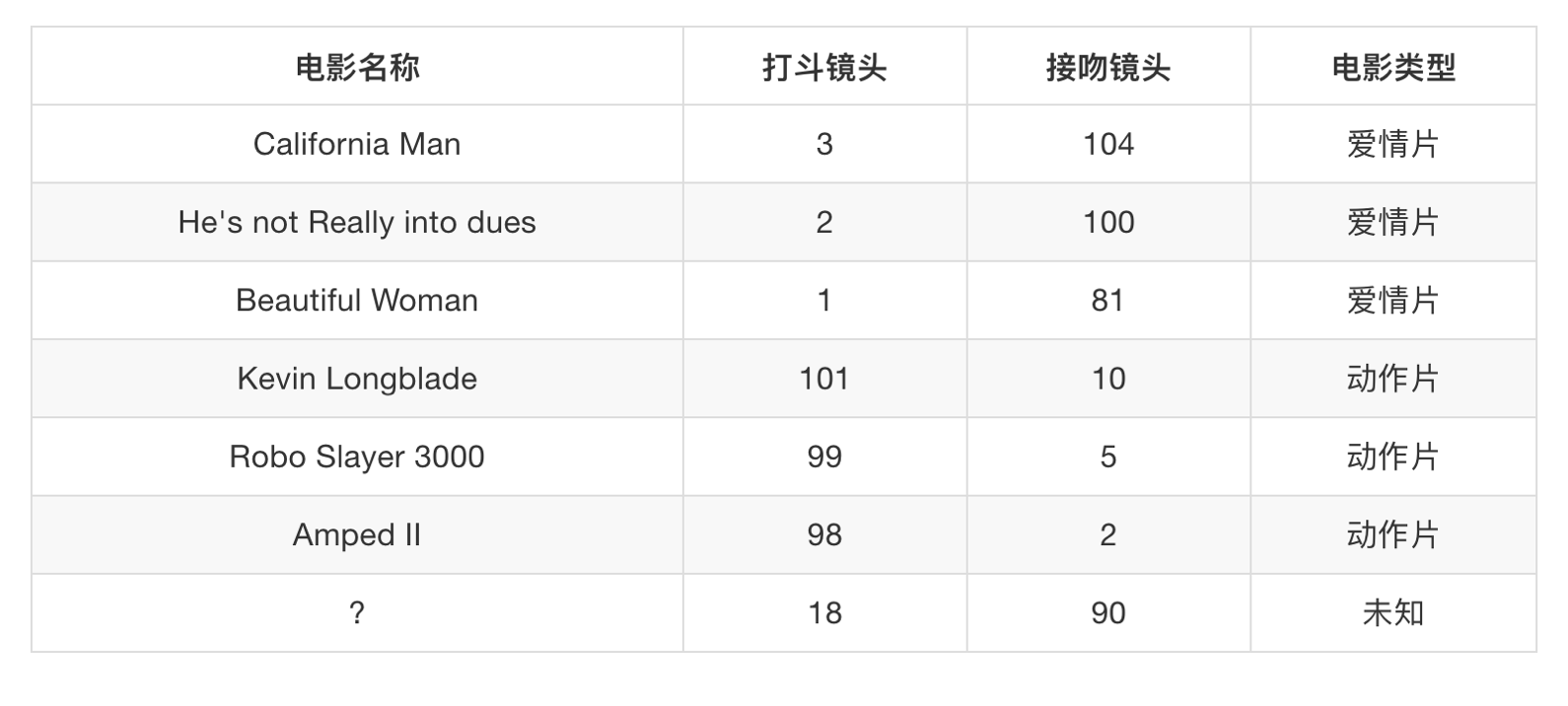其中？ 号电影不知道类别，如何去预测？我们可以利用K近邻算法的思想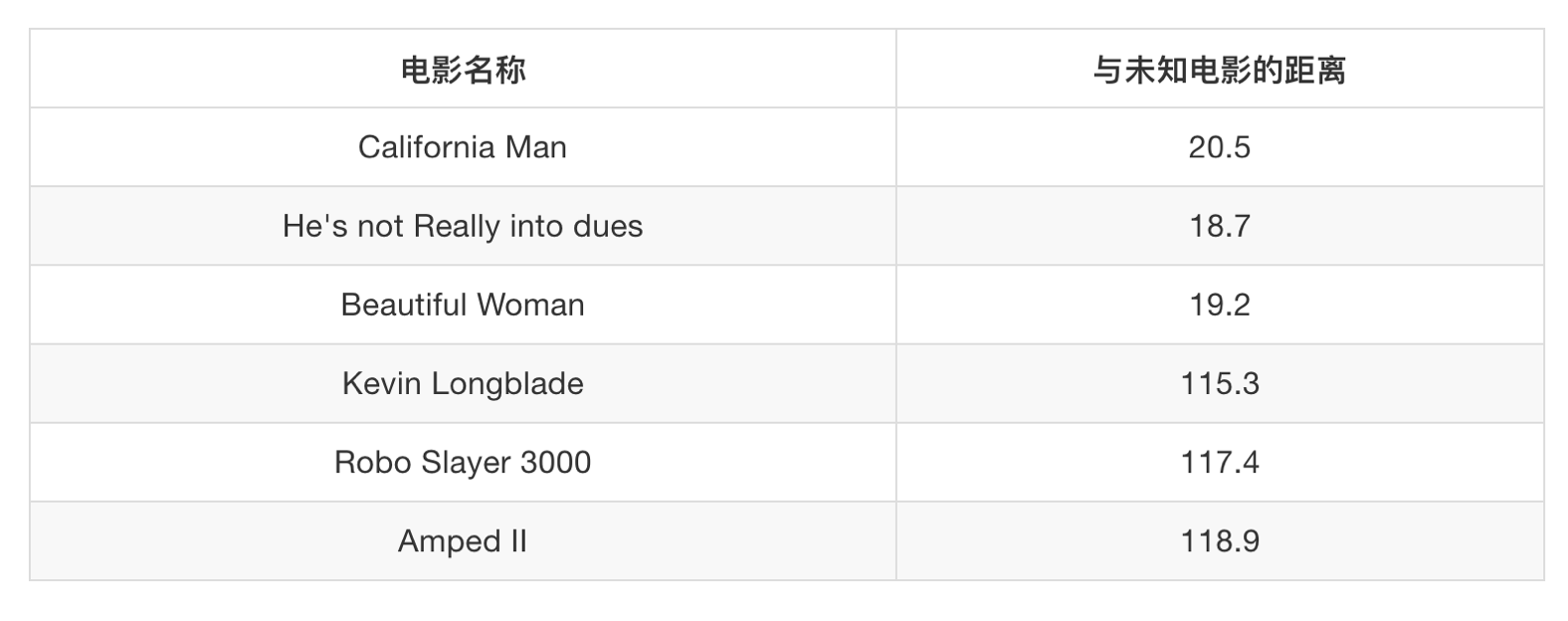用?的特征值,和其它6部电影计算距离.eg:(3-18)^ 2 +(104-90)^ 2=20.5
3.3 K-近邻算法API
sklearn.neighbors.KNeighborsClassifier(n_neighbors=5,algorithm=‘auto’)
n_neighbors：int,可选（默认= 5），k_neighbors查询默认使用的邻居数
algorithm：{‘auto’，‘ball_tree’，‘kd_tree’，‘brute’}，可选用于计算最近邻居的算法：‘ball_tree’将会使用 BallTree，‘kd_tree’将使用 KDTree。‘auto’将尝试根据传递给fit方法的值来决定最合适的算法。 (不同实现方式影响效率)
3.4 案例：预测签到位置
kaggle大赛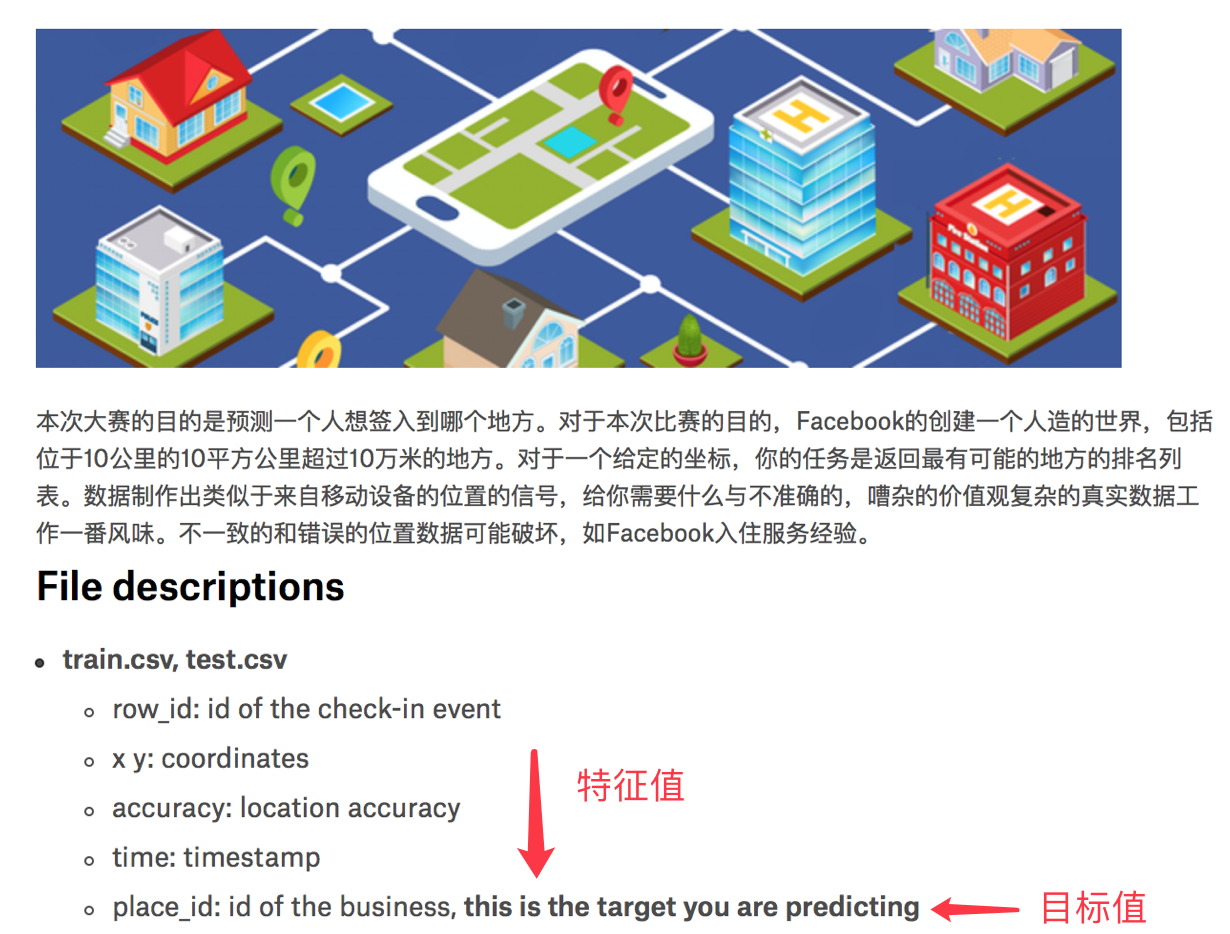数据介绍：
train.csv，test.csv
row_id：登记事件的ID
xy：坐标
准确性：定位准确性
时间：时间戳
place_id：业务的ID，这是您预测的目标

3.4.1 分析
对于数据做一些基本处理（这里所做的一些处理不一定达到很好的效果，我们只是简单尝试，有些特征我们可以根据一些特征选择的方式去做处理）
1、缩小数据集范围 DataFrame.query()
2、处理日期数据 pd.to_datetime pd.DatetimeIndex
3、增加分割的日期数据
4、删除没用的日期数据 DataFrame.drop
5、将签到位置少于n个用户的删除
place_count = data.groupby(‘place_id’).count()
tf = place_count[place_count.row_id > 3].reset_index()
data = data[data[‘place_id’].isin(tf.place_id)]
3.4.2 代码
from sklearn.preprocessing import StandardScaler
from sklearn.neighbors import KNeighborsClassifier
from sklearn.model_selection import train_test_split
import pandas as pd

def knncls():
'''
K-近邻算法预测用户查询的业务
:return:
'''
data = pd.read_csv("./data/facebook-v-predicting-check-ins/train.csv")
#print(data)
#1.缩小数据范围
data = data.query('x>1.0 & x<1.25 & y>2.5 & y<2.75')

#3.把签到位置小于N个人的位置给删除掉
place_count = data.groupby('place_id').count()

tf = place_count[place_count.row_id > 3].reset_index()

data = data[data['place_id'].isin(tf.place_id)]

#4.分割数据集到训练集和测试集
#取出特征值和目标值
#y = data[['place_id']]            #目标值
y = data['place_id'] #一维数据
x = data[['x', 'y', 'accuracy', 'time']]
x_train, x_test ,y_train, y_test = train_test_split(x, y, test_size=0.3)

#进行数据的标准化处理,测试集和训练集分开标准化
std = StandardScaler()

#对训练集的特征值作标准化处理
x_train = std.fit_transform(x_train)

#对测试集的特征值作标准化处理
x_test = std.fit_transform(x_test)
#5应用网格搜索+交叉验证-K近邻算法去进行调优
knn = KNeighborsClassifier(n_neighbors=5)

#利用fit和predict或者score
knn.fit(x_train, y_train)

#预测测试集的所办业务类型
y_pridict = knn.predict(x_test)
print("k近邻算法预测的这些时间的业务类型", y_pridict)
print("k近邻预测的准确率为：", knn.score(x_test, y_test))
return None

if __name__=='__main__':
knncls()


输出结果:
k近邻算法预测的这些时间的业务类型 [6399991653 5606572086 6399991653 ... 9237487147 6424972551 8780655195]
k近邻预测的准确率为： 0.5120173364854216
Process finished with exit code 0

3.4.3 结果分析
1、k值取多大？有什么影响？
k值取很小：容易受到异常点的影响
k值取很大：受到样本均衡的问题
2、性能问题？
距离计算上面，时间复杂度高
3.5 总结
优点：
简单，易于理解，易于实现，无需训练
缺点：
懒惰算法，对测试样本分类时的计算量大，内存开销大
必须指定K值，K值选择不当则分类精度不能保证
使用场景：小数据场景，几千～几万样本，具体场景具体业务去测试
4 模型选择与调优
目标
说明交叉验证过程
说明超参数搜索过程
应用GridSearchCV实现算法参数的调优
应用
Facebook签到位置预测调优
4.1 为什么需要交叉验证
交叉验证目的：为了让被评估的模型更加准确可信.
4.2 什么是交叉验证(cross validation)
交叉验证：将拿到的训练数据，分为训练和验证集。以下图为例：将数据分成5份，其中一份作为验证集。然后经过5次(组)的测试，每次都更换不同的验证集。即得到5组模型的结果，取平均值作为最终结果。又称5折交叉验证。
分析:
我们之前知道数据分为训练集和测试集，但是为了让从训练得到模型结果更加准确。做以下处理
训练集：训练集+验证集
测试集：测试集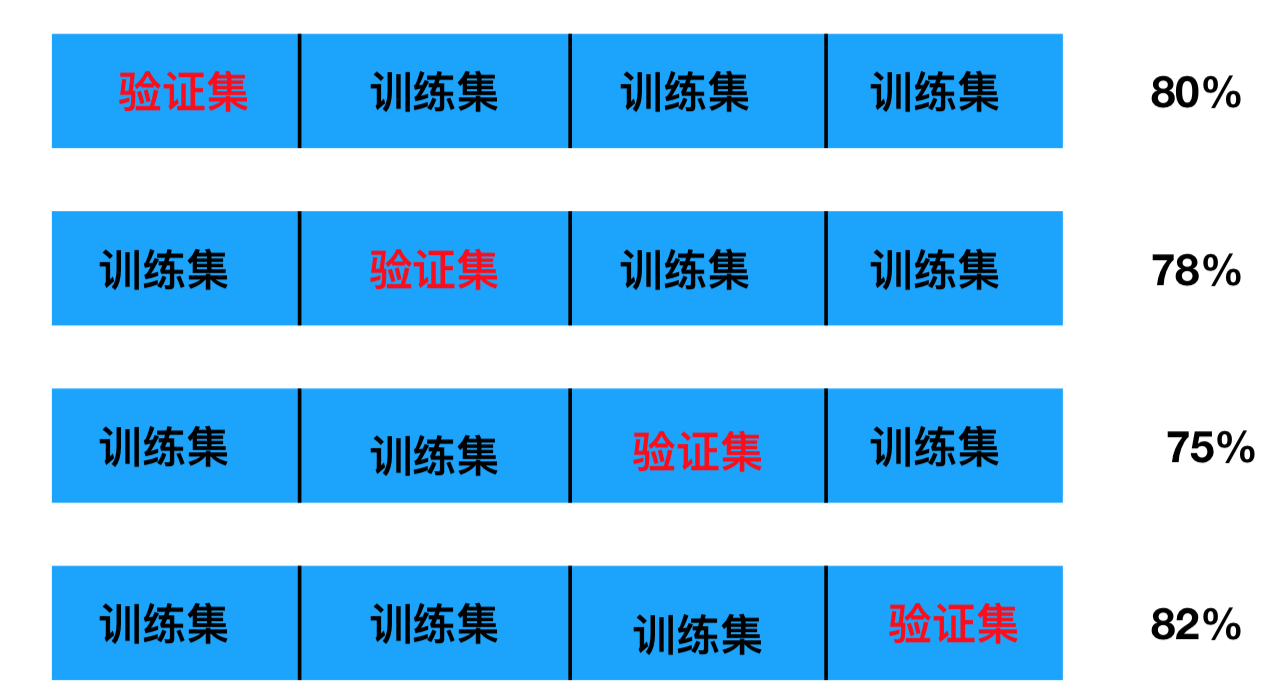问题:如何给模型选择最好的参数呢?
4.3 超参数搜索-网格搜索(Grid Search)
通常情况下，有很多参数是需要手动指定的（如k-近邻算法中的K值），这种叫超参数。但是手动过程繁杂，所以需要对模型预设几种超参数组合。每组超参数都采用交叉验证来进行评估。最后选出最优参数组合建立模型。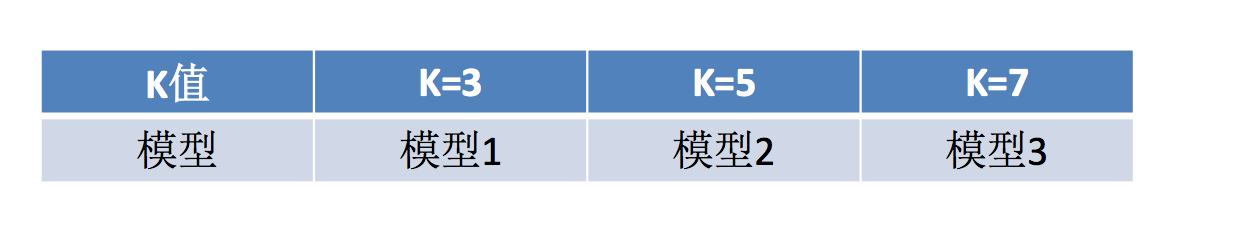API:
sklearn.model_selection.GridSearchCV(estimator, param_grid=None,cv=None)
对估计器的指定参数值进行详尽搜索
estimator：估计器对象
param_grid：估计器参数(dict){“n_neighbors”:[1,3,5]}
cv：指定几折交叉验证
fit：输入训练数据
score：准确率
结果分析：
bestscore:在交叉验证中验证的最好结果
bestestimator：最好的参数模型
cvresults:每次交叉验证后的验证集准确率结果和训练集准确率结果
4.4 Facebook签到位置预测K值调优
代码:
from sklearn.model_selection import GridSearchCV
from sklearn.preprocessing import StandardScaler
from sklearn.neighbors import KNeighborsClassifier
from sklearn.model_selection import train_test_split
import pandas as pd

def knncls():
'''
K-近邻算法预测用户查询的业务
:return:
'''
data = pd.read_csv("./data/facebook-v-predicting-check-ins/train.csv")
#print(data)
#1.缩小数据范围
data = data.query('x>1.0 & x<1.25 & y>2.5 & y<2.75')

#3.把签到位置小于N个人的位置给删除掉
place_count = data.groupby('place_id').count()

tf = place_count[place_count.row_id > 3].reset_index()

data = data[data['place_id'].isin(tf.place_id)]

#4.分割数据集到训练集和测试集
#取出特征值和目标值
#y = data[['place_id']]  #目标值,这样写y是二维数据,knn允许训练,但gc不行,所以写成1维数据
y = data['place_id'] #一维数据
x = data[['x', 'y', 'accuracy', 'time']]
x_train, x_test ,y_train, y_test = train_test_split(x, y, test_size=0.3)

#进行数据的标准化处理,测试集和训练集分开标准化
std = StandardScaler()

#对训练集的特征值作标准化处理
x_train = std.fit_transform(x_train)

#对测试集的特征值作标准化处理
x_test = std.fit_transform(x_test)
#5应用网格搜索+交叉验证-K近邻算法去进行调优
knn = KNeighborsClassifier(n_neighbors=5)

#构造超参数字典
#对knn来讲，数据量比较大,k =根号（样本）
param = {"n_neighbors": [1, 3, 5, 7, 10]}

#估计器是knn;为了看到效果，cv = 2, 通常会选择10
gc = GridSearchCV(knn, param_grid=param, cv=2)

#gc想当于被包裹的estimator,fit输入数据,进行训练
gc.fit(x_train, y_train)

#查看模型超参数调优的过程，交叉验证的结果
print("在2折交叉验证当中最好的结果：",gc.best_score_)
print("选择的最好的模型参数是：", gc.best_estimator_)
print("每次交叉验证的验证集的预测结果：", gc.cv_results_)

#预测测试集的准确率
print("在测试集当中的最终预测结果为：", gc.score(x_test, y_test))

return None

if __name__=='__main__':
knncls()

输出结果:
交叉验证当中最好的结果： 0.5160445870629962
选择的最好的模型参数是： KNeighborsClassifier(algorithm='auto', leaf_size=30, metric='minkowski',
metric_params=None, n_jobs=None, n_neighbors=10, p=2,
weights='uniform')
每次交叉验证的验证集的预测结果： {
'mean_test_score': array([0.44983955, 0.47829758, 0.5052356 , 0.51418679, 0.51604459]),
'param_n_neighbors': masked_array(data=[1, 3, 5, 7, 10], dtype=object),
'split1_train_score': array([1.        , 0.66376715, 0.6239545 , 0.6022081 , 0.57176313]),
'split0_train_score': array([1.        , 0.67360164, 0.62653479, 0.6026603 , 0.58475443]),
'split1_test_score': array([0.45310368, 0.48209413, 0.51432469, 0.52353342, 0.52557981]),
'split0_test_score': array([0.44663767, 0.47457344, 0.49631984, 0.5050184 , 0.5066912 ]), .........................dtype=int32)}
在测试集当中的最终预测结果为： 0.5486603624901497

Process finished with exit code 0

5.朴素贝叶斯算法
学习目标:
说明条件概率与联合概率
说明贝叶斯公式、以及特征独立的关系
记忆贝叶斯公式
知道拉普拉斯平滑系数
应用贝叶斯公式实现概率的计算
应用
20类新闻文章分类预测
5.1 什么是朴素贝叶斯分类方法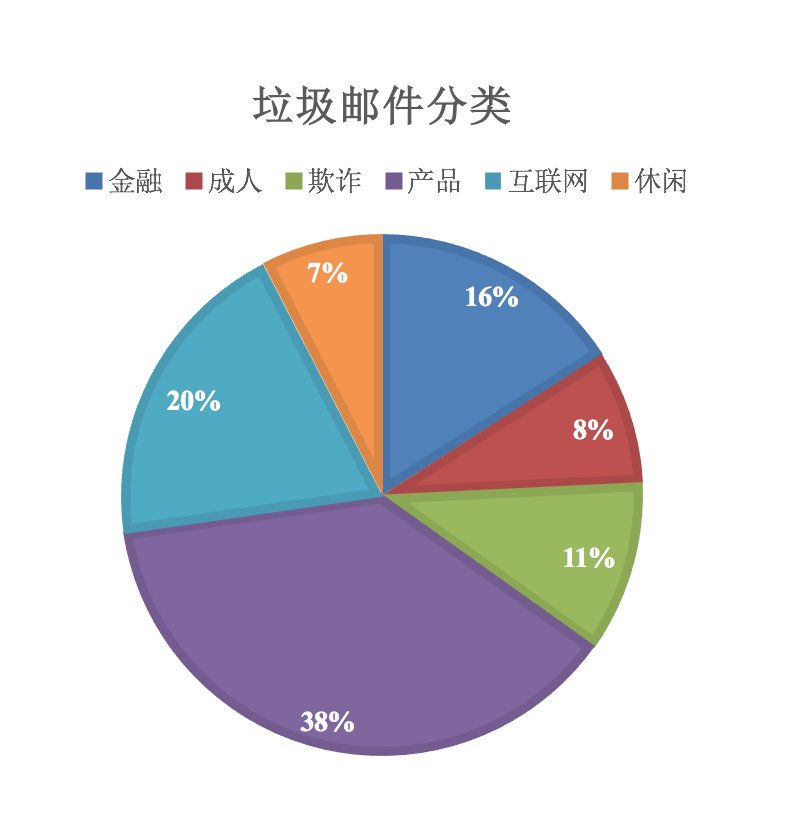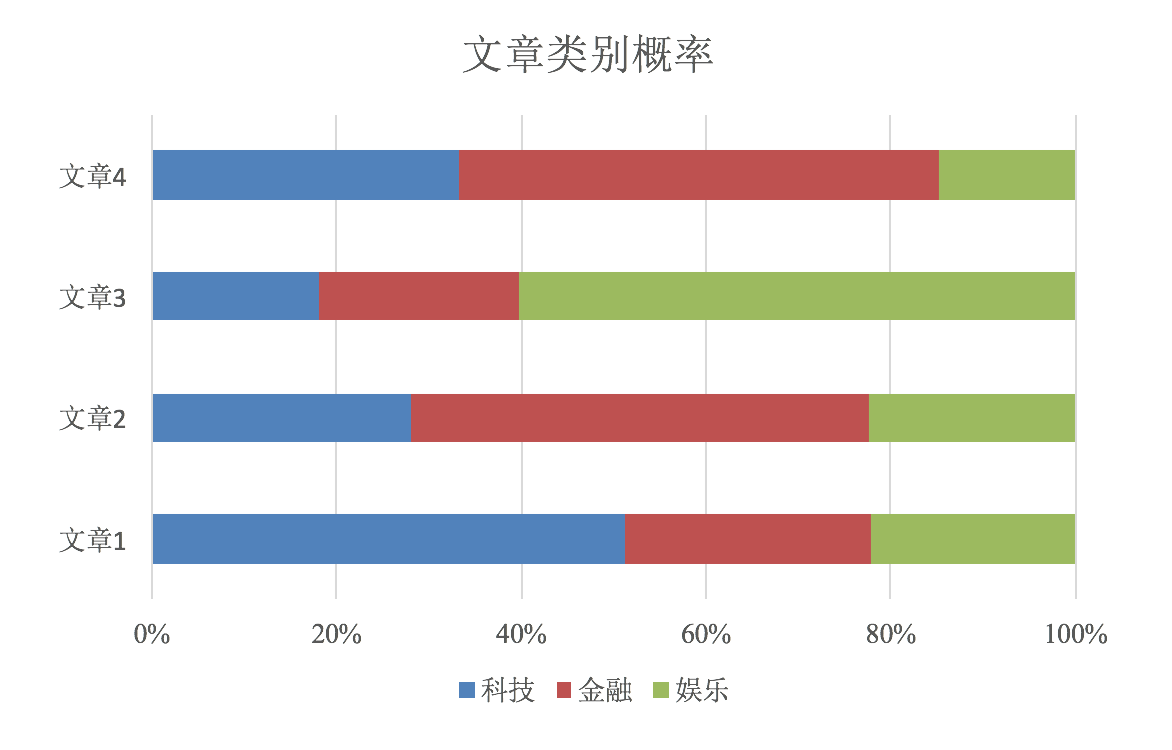5.2 概率基础
5.2.1 概率(Probability)定义
概率定义为一件事情发生的可能性
扔出一个硬币，结果头像朝上
某天是晴天
P(X) : 取值在[0, 1]
5.2.2 女神是否喜欢计算案例
在讲这两个概率之前我们通过一个例子，来计算一些结果：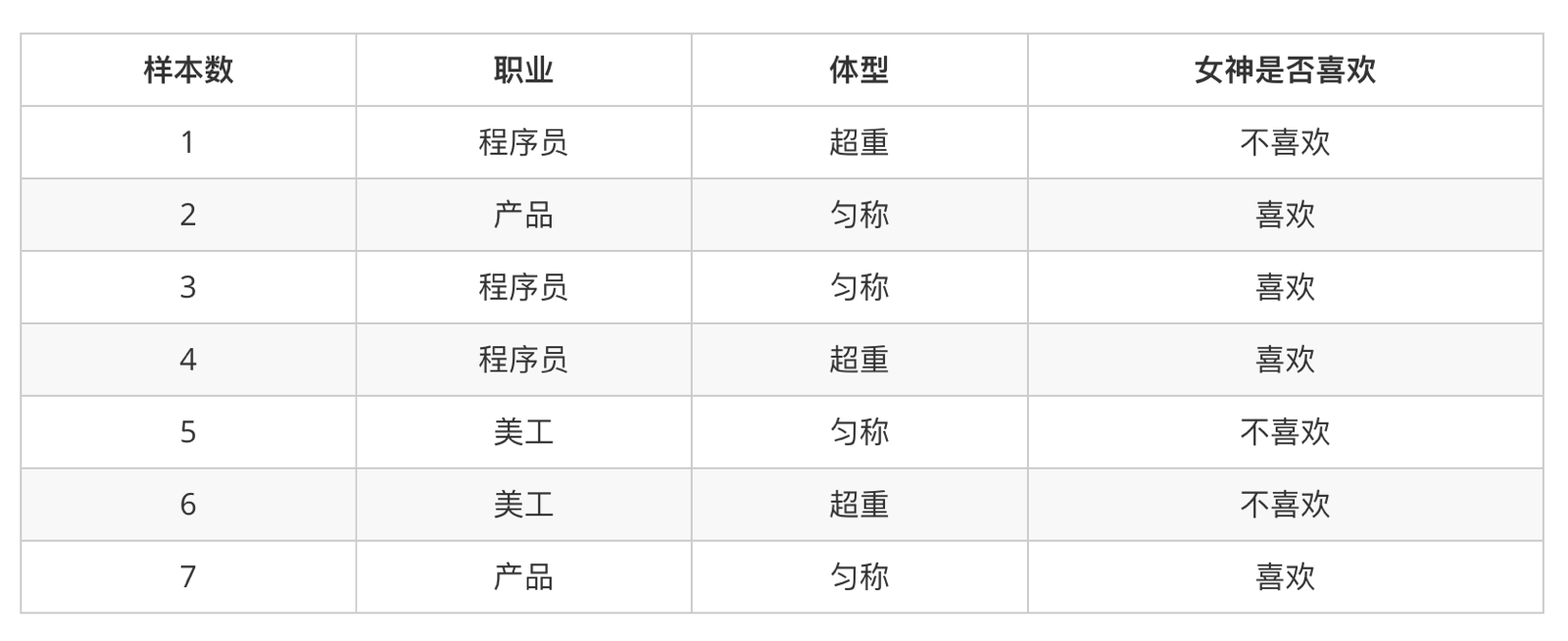问题如下：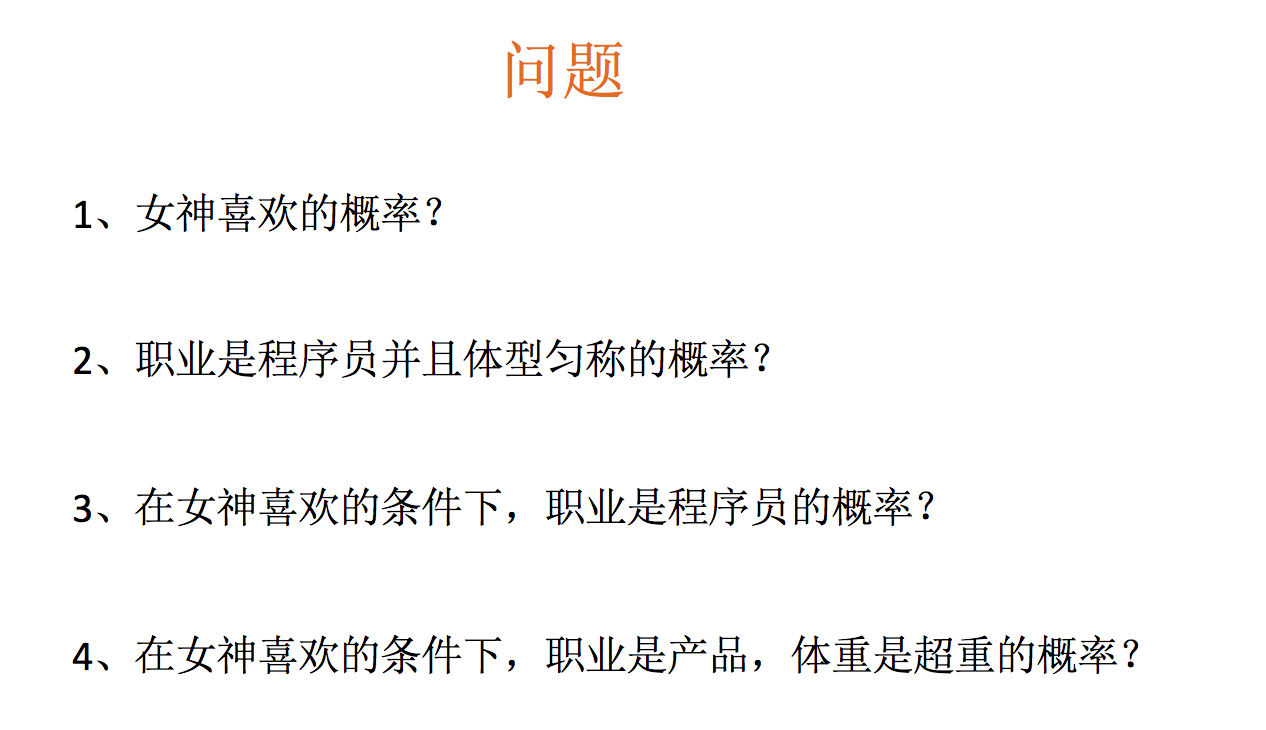P(喜欢) = 4/7
P(程序员, 体型匀称) = P(程序员)P(体型匀称)=3/7 * 4/7  =12/49
P(程序员|喜欢) = 1/2
P(产品,超重|喜欢) = 1/2 * 1/4 = 1/8

5.2.3 条件概率与联合概率
联合概率：包含多个条件，且所有条件同时成立的概率
记作：P(A,B)
特性：P(A, B) = P(A)P(B)
条件概率：就是事件A在另外一个事件B已经发生条件下的发生概率
记作：P(A|B) = P(AB)/P(B)
特性：P(A1,A2|B) = P(A1|B)P(A2|B)
注意：此条件概率的成立，是由于A1,A2相互独立的结果(记忆)

这样我们计算结果为：
p(程序员, 匀称) =  P(程序员)P(匀称) =3/7*(4/7) = 12/49
P(产品, 超重|喜欢) = P(产品|喜欢)P(超重|喜欢)=1/2 *  1/4 = 1/8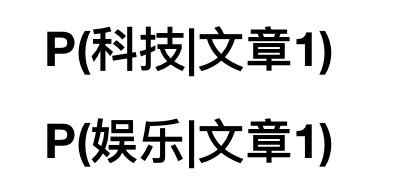这个了类似一个条件概率，那么仔细一想，给定文章其实相当于给定什么？结合前面我们将文本特征抽取的时候讲的？所以我们可以理解为: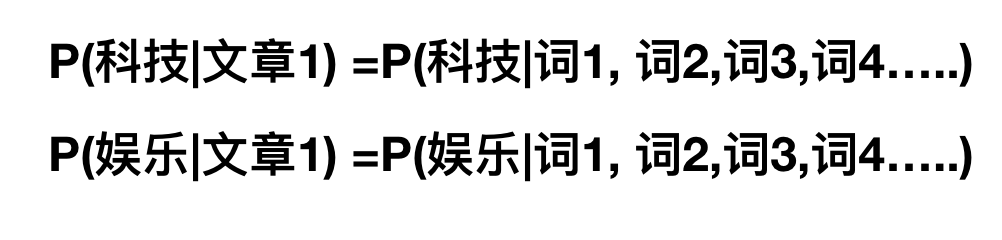5.3 贝叶斯公式
5.3.1 公式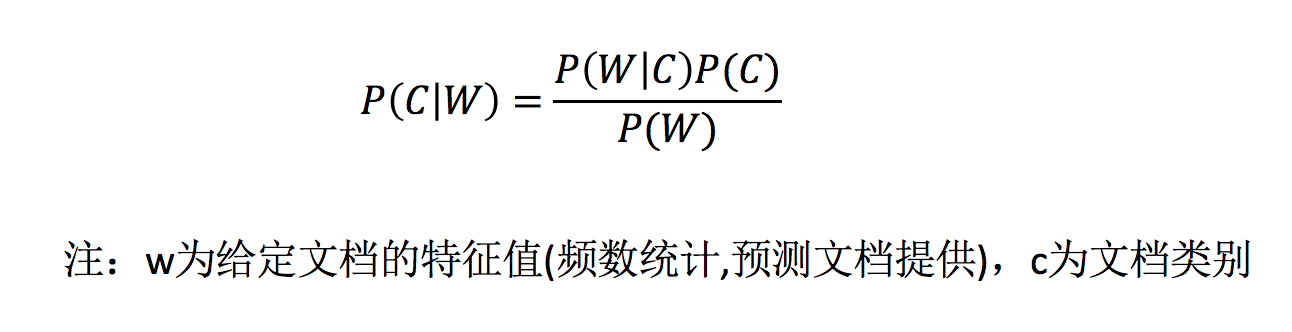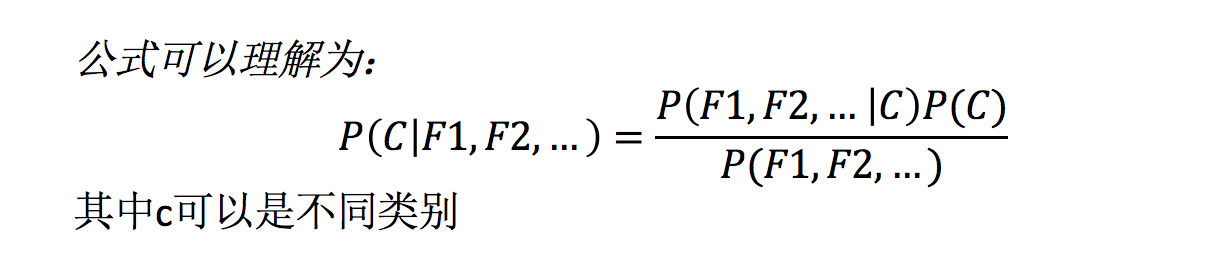公式分为三个部分：
P©：每个文档类别的概率(某文档类别数／总文档数量)
P(W│C)：给定类别下特征（被预测文档中出现的词）的概率
计算方法：P(F1│C)=Ni/N （训练文档中去计算）
Ni为该F1词在C类别所有文档中出现的次数
N为所属类别C下的文档所有词出现的次数和
P(F1,F2,…) 预测文档中每个词的概率
P(科技|文章1) = P(词1, 词2,词3....词n|科技)P(科技)/P((词1, 词2,词3....词n)
P(娱乐|文章1) = P(词1, 词2,词3....词n|娱乐)P(娱乐))/P((词1, 词2,词3....词n)

5.3.2 文章分类计算
假设我们从训练数据集得到如下信息: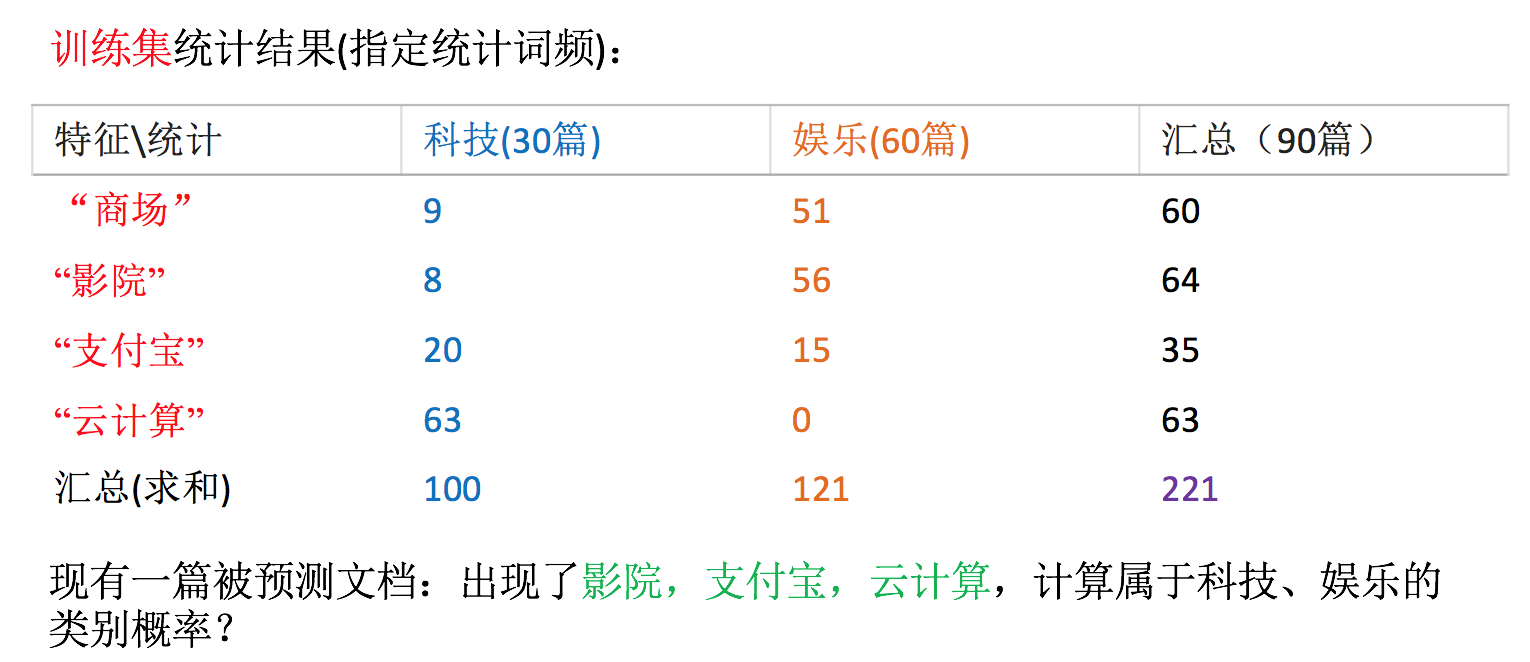计算结果:
科技：P(科技|影院,支付宝,云计算) =
?(影院,支付宝,云计算|科技)∗P(科技)=
(8/100)∗(20/100)∗(63/100)∗(30/90) = 0.00456109

娱乐：P(娱乐|影院,支付宝,云计算) =
?(影院,支付宝,云计算|娱乐)∗P(娱乐)=
(56/121)∗(15/121)∗(0/121)∗(60/90) = 0

思考:我们计算出来某个概率为0，合适吗？
5.3.3 拉普拉斯平滑系数
目的：防止计算出的分类概率为0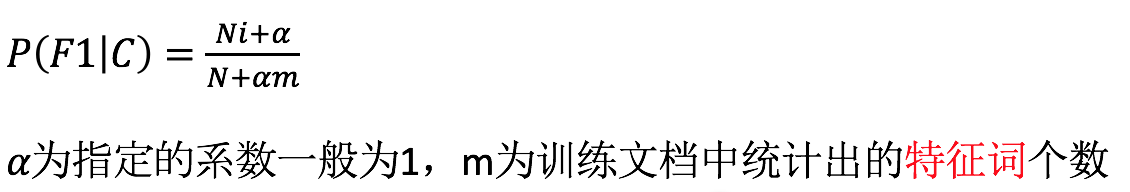P(娱乐|影院,支付宝,云计算) =
P(影院,支付宝,云计算|娱乐)P(娱乐) =
P(影院|娱乐)*P(支付宝|娱乐)*P(云计算|娱乐)P(娱乐)=
(56+1/121+4)(15+1/121+4)(0+1/121+1*4)(60/90) = 0.00002

5.3.4 API
sklearn.naive_bayes.MultinomialNB(alpha = 1.0)
朴素贝叶斯分类
alpha：拉普拉斯平滑系数
5.4 案例：20类新闻分类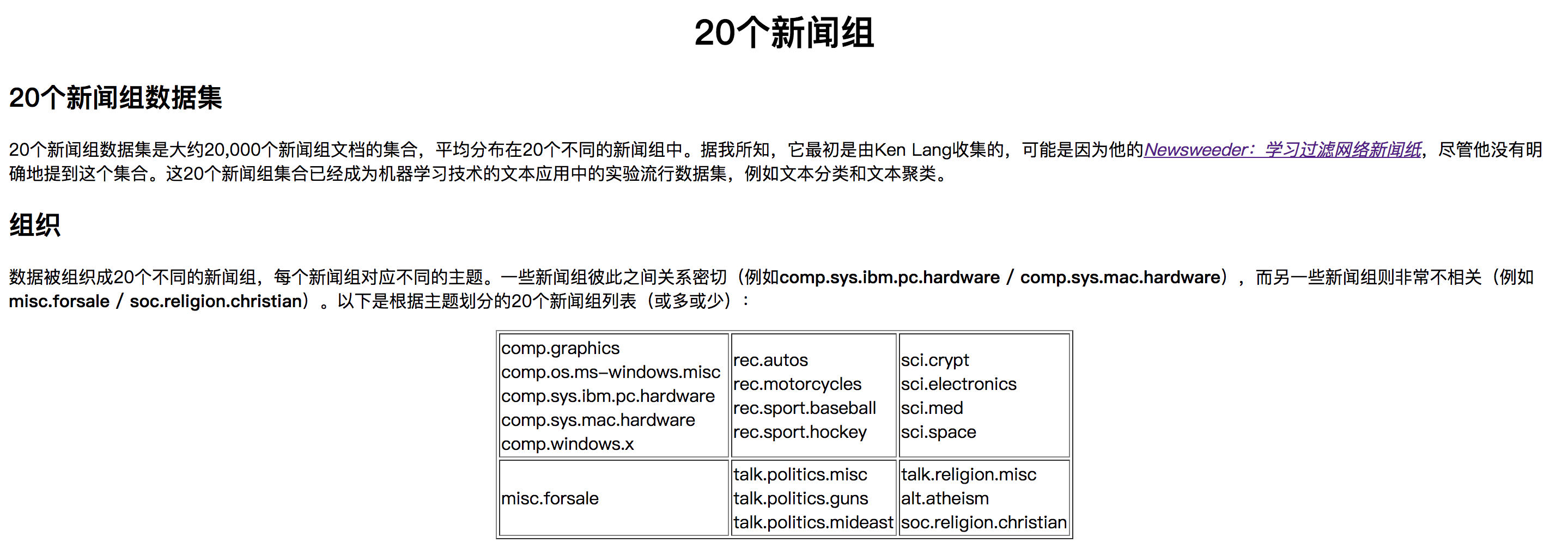5.4.1 分析
分割数据集
tfidf进行的特征抽取
朴素贝叶斯预测
5.4.2 代码
from sklearn.datasets import fetch_20newsgroups
from sklearn.model_selection import train_test_split
from sklearn.feature_extraction.text import TfidfVectorizer
from sklearn.naive_bayes import MultinomialNB

def nbcls():
"""
朴素贝叶斯对新闻数据集进行预测
:return:
"""
# 获取新闻的数据，20个类别,包含特征值和目标值
news = fetch_20newsgroups(subset='all')

# 进行数据集分割
x_train, x_test, y_train, y_test = train_test_split(news.data, news.target, test_size=0.3)

# 对于文本数据，进行特征抽取
tfidf = TfidfVectorizer()

x_train = tfidf.fit_transform(x_train)
# 这里打印出来的列表是：训练集当中的所有不同词的组成的一个列表
a = tfidf.get_feature_names()
# print(x_train.toarray())

# 不能调用fit_transform,否则会出错,用x_train去转换,因为预测文档是基于训练集预测的
x_test = tfidf.transform(x_test)
b = tfidf.get_feature_names()

#print(a==b) #a!=b
# estimator估计器流程
mlb = MultinomialNB(alpha=1.0)

mlb.fit(x_train, y_train)

# 进行预测
y_predict = mlb.predict(x_test)

print("预测每篇文章的类别：", y_predict[:50]) #打印前50个
print("真实类别为：", y_test[:50])      #打印前50个

print("预测准确率为：", mlb.score(x_test, y_test))

return None

if __name__=='__main__':
nbcls()

输出结果:
预测每篇文章的类别： [11 10  8 13 13 13  0  7 11  7  2  7 10 13 10  9 15  9 16  4 17 16 11 10
2  0  9  2 10  3 14  4 14 14 12 14 18  8  0  6  6  2  4  4  2 13 11 13
10 15]
真实类别为： [11 10  8 13 13  5  0  7 11  7  2  7 10 13 10  9 15  9 16  4 17 19 12 10
4  0  9  2 10  3 14  4 14 14  3 14 18  8  0  6  6  2  4  4  5 13 11 13
10 11]
预测准确率为： 0.8542624690484613

Process finished with exit code 0

5.5 总结
优点：
朴素贝叶斯模型发源于古典数学理论，有稳定的分类效率。
对缺失数据不太敏感，算法也比较简单，常用于文本分类。
分类准确度高，速度快
缺点：
由于使用了样本属性独立性的假设，所以如果特征属性有关联时其效果不好
6.决策树
目标:
说明信息熵的公式以及作用
说明信息增益的公式作用
应用信息增益实现计算特征的不确定性减少程度
了解决策树的三种算法实现
应用:
泰坦尼克号乘客生存预测
怎么理解这句话？通过一个对话例子
6.1 认识决策树
决策树思想的来源非常朴素，程序设计中的条件分支结构就是if-then结构，最早的决策树就是利用这类结构分割数据的一种分类学习方法
怎么理解这句话？通过一个对话例子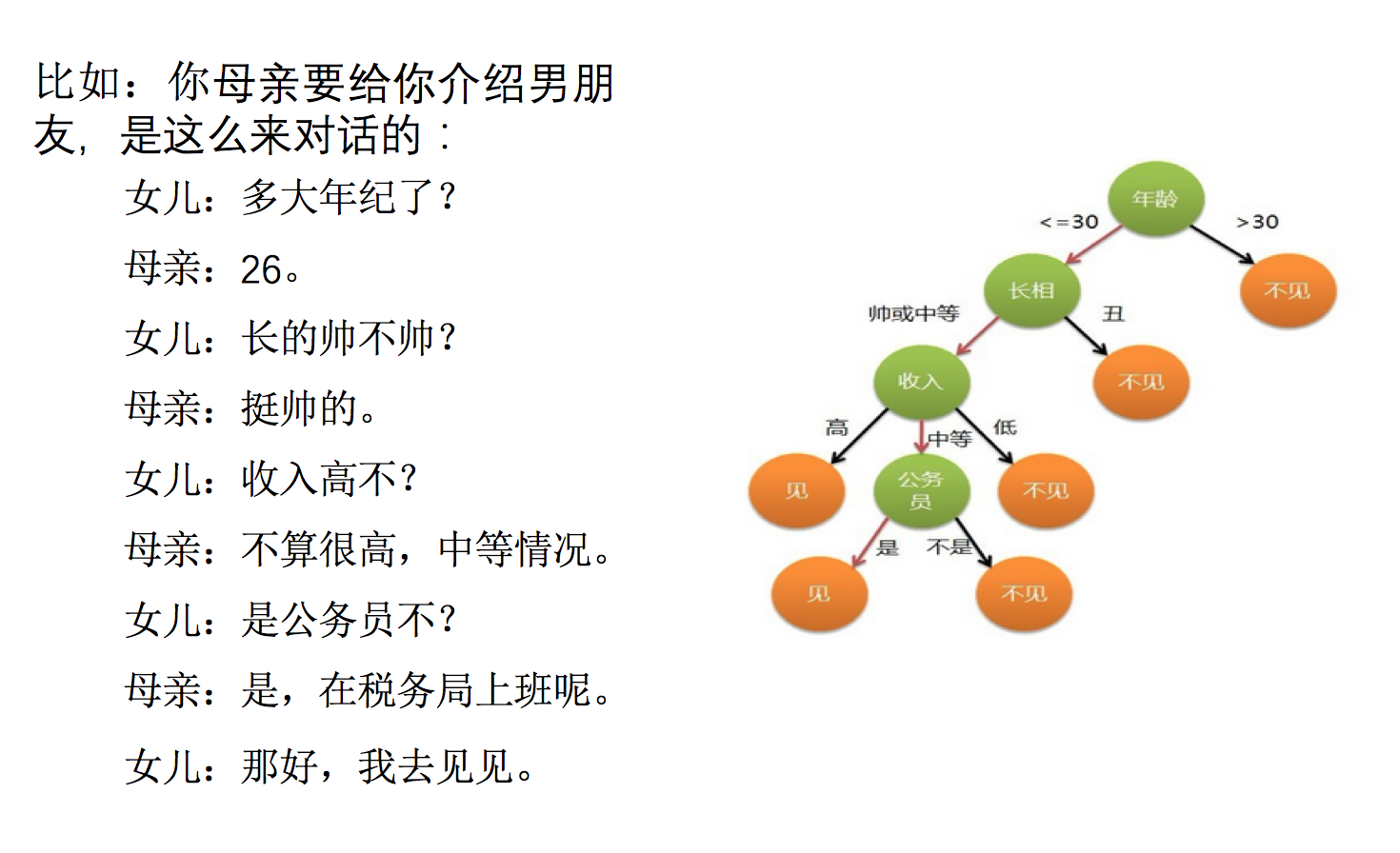想一想这个女生为什么把年龄放在最上面判断！！！！！！！！！

6.2 决策树分类原理详解
为了更好理解决策树具体怎么分类的，我们通过一个问题例子？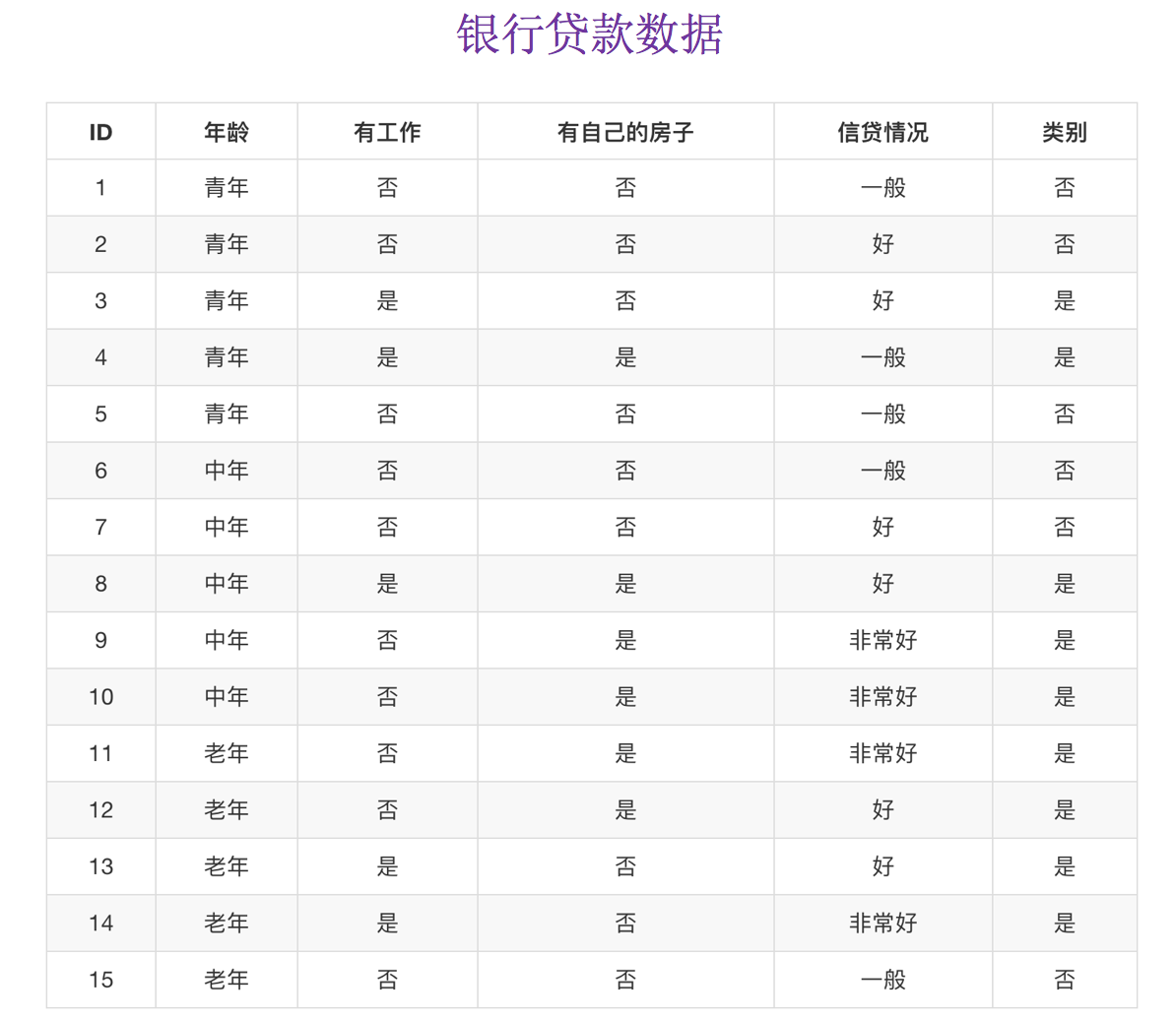问题：如何对这些客户进行分类预测？你是如何去划分？
有可能你的划分是这样的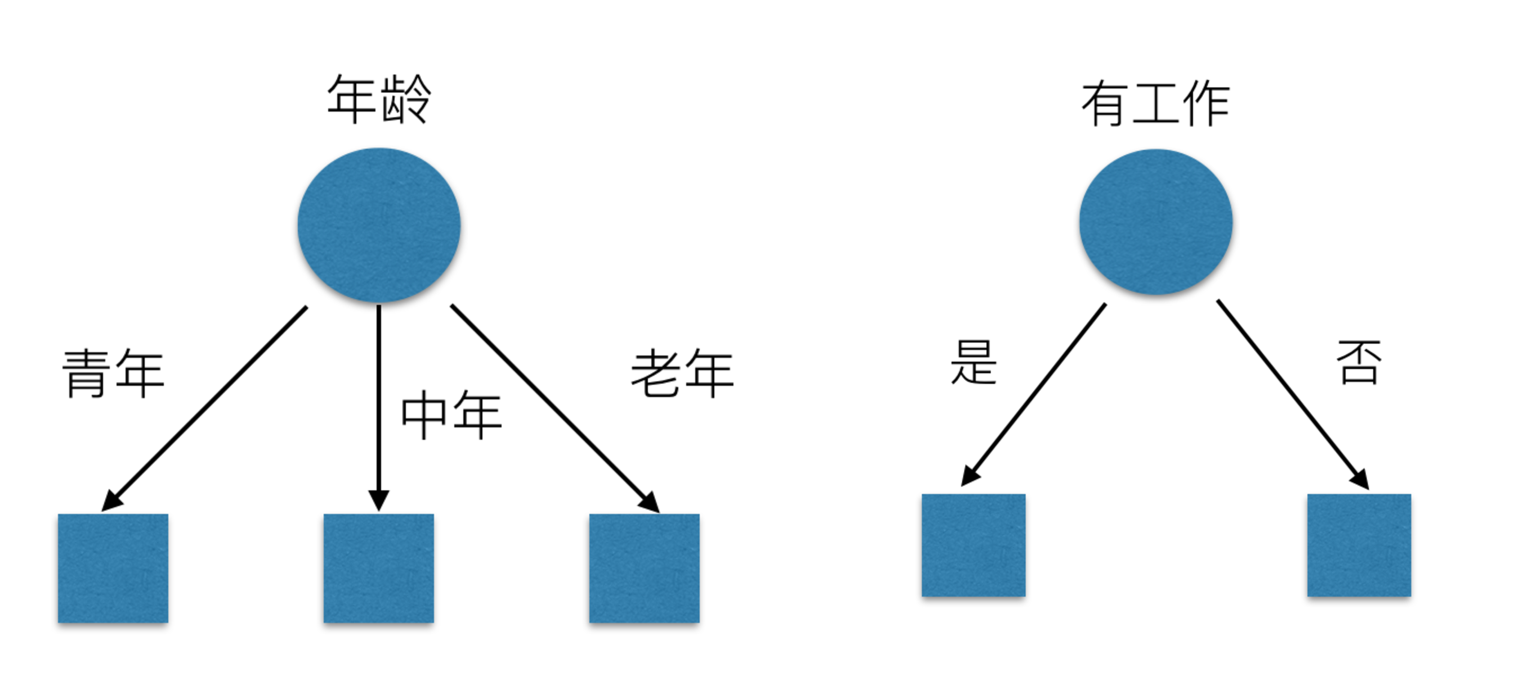那么我们怎么知道这些特征哪个更好放在最上面，那么决策树的真是划分是这样的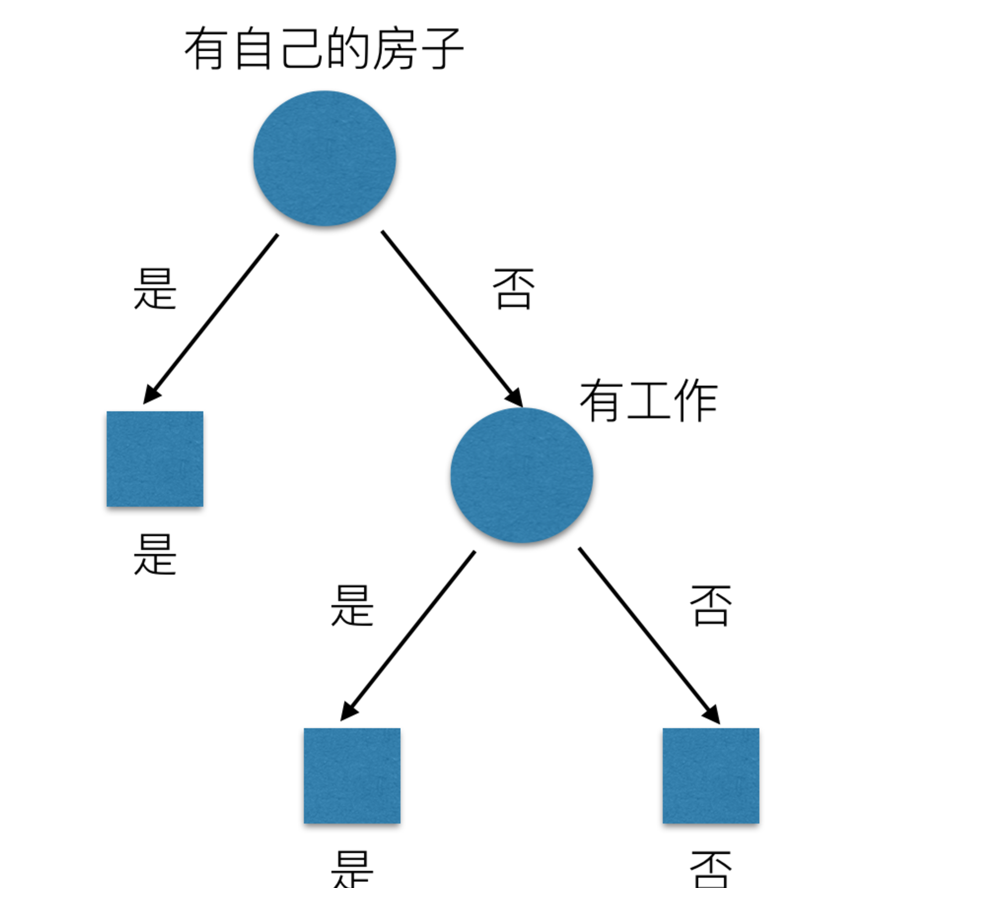6.2.1 原理
信息熵、信息增益等
6.2.2 信息熵
那来玩个猜测游戏，猜猜这32支球队那个是冠军。并且猜测错误付出代价。每猜错一次给一块钱，告诉我是否猜对了，那么我需要掏多少钱才能知道谁是冠军？ （前提是：不知道任意球队的信息、历史比赛记录、实力等）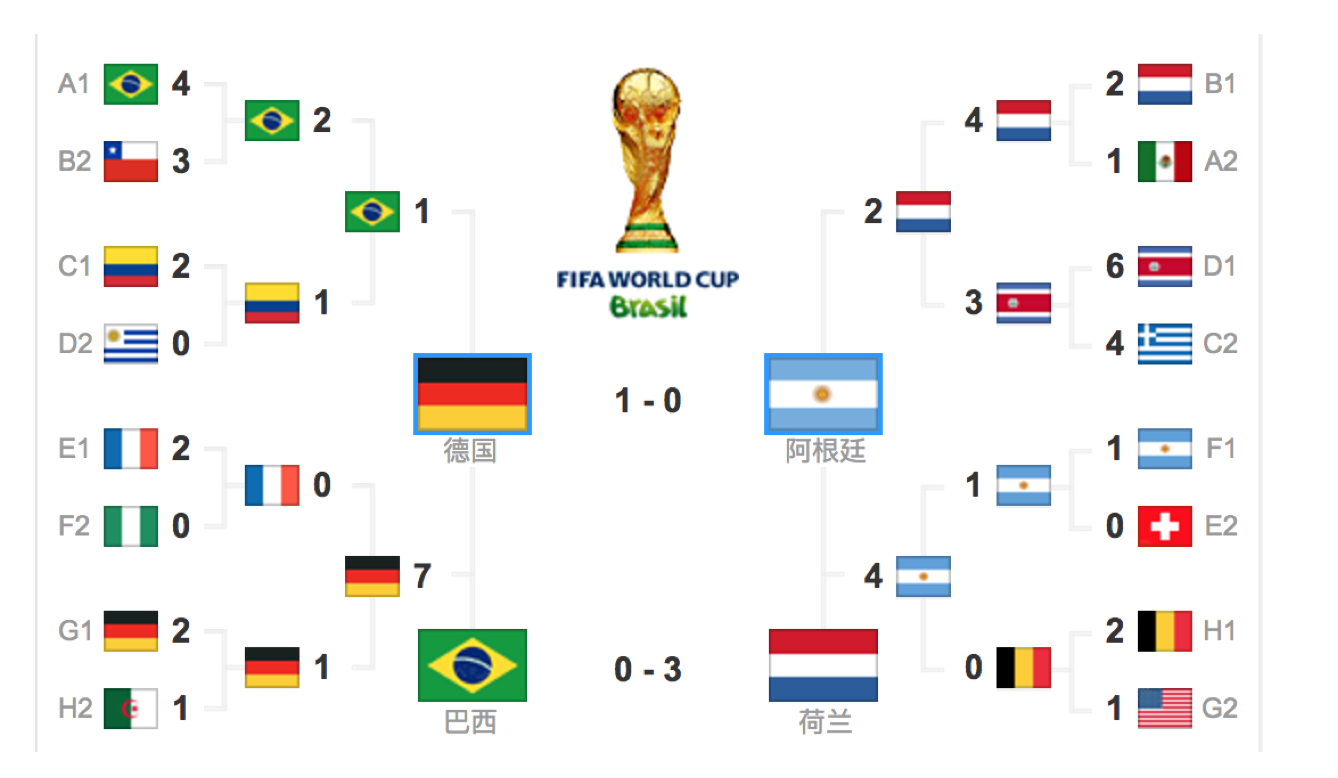为了使代价最小，可以使用二分法猜测：
我可以把球编上号，从1到32，然后提问：冠 军在1-16号吗？依次询问，只需要五次，就可以知道结果。我们来看这个式子：
32支球队，log32=5比特
64支球队，log64=6比特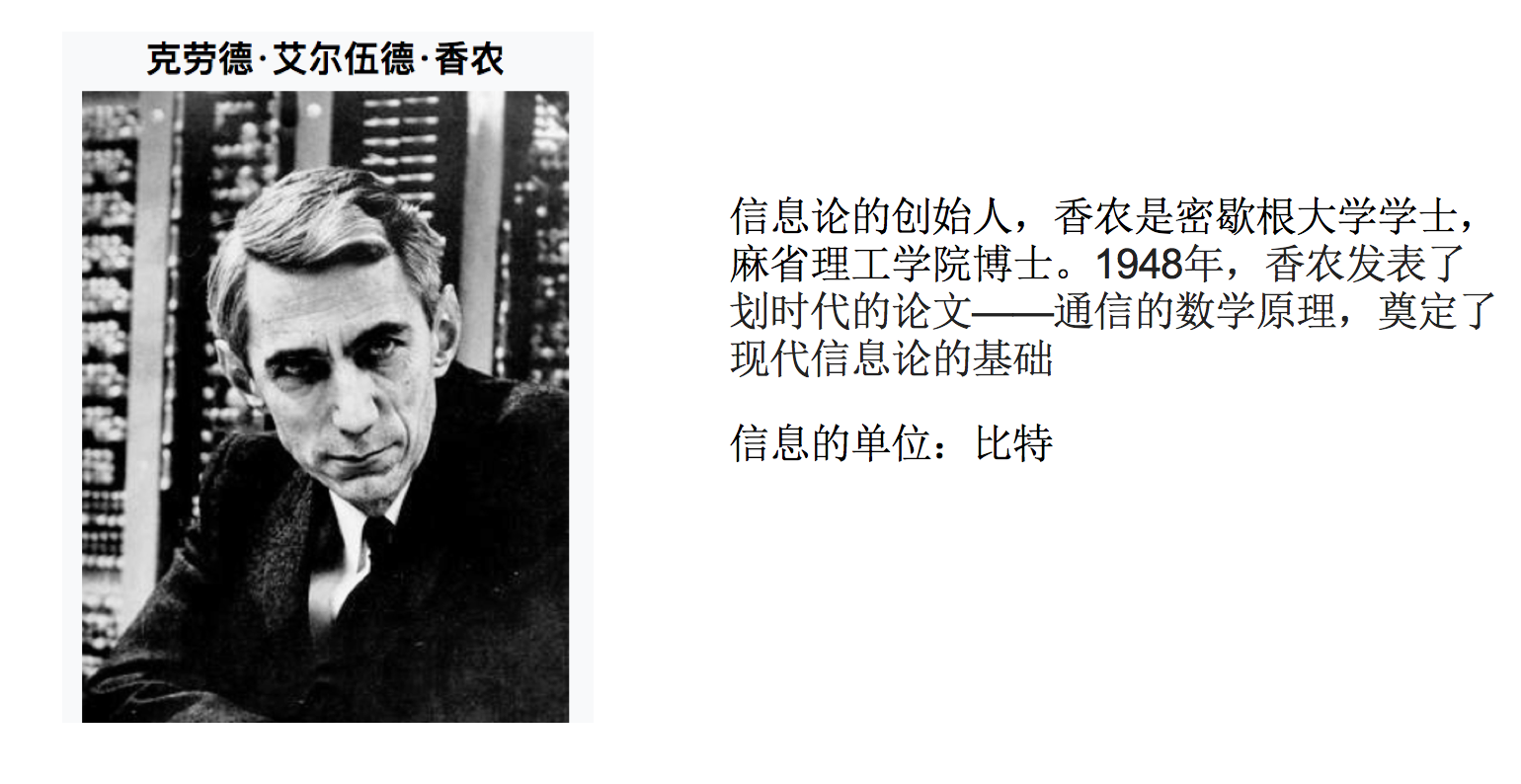香农指出，它的准确信息量应该是，p为每个球队获胜的概率（假设概率相等，都为1/32），我们不用钱去衡量这个代价了，香浓指出用比特：
H = -(p1logp1 + p2logp2 + ... + p32log32) = - log32

6.2.2.1 信息熵的定义
H的专业术语称之为信息熵，单位为比特。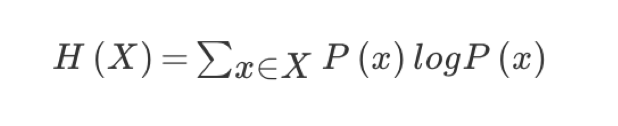“谁是世界杯冠军”的信息量应该比5比特少，特点（重要）：
当这32支球队夺冠的几率相同时，对应的信息熵等于5比特
只要概率发生任意变化，信息熵都比5比特大
6.2.2.2 总结（重要）
信息和消除不确定性是相联系的
当我们得到的额外信息（球队历史比赛情况等等）越多的话，那么我们猜测的代价越小（猜测的不确定性减小）
问题： 回到我们前面的贷款案例，怎么去划分？可以利用当得知某个特征（比如是否有房子）之后，我们能够减少的不确定性大小。越大我们可以认为这个特征很重要。那怎么去衡量减少的不确定性大小呢？
6.2.3 决策树的划分依据之一------信息增益
6.2.3.1 定义与公式
特征A对训练数据集D的信息增益g(D,A),定义为集合D的信息熵H(D)与特征A给定条件下D的信息条件熵H(D|A)之差，即公式为：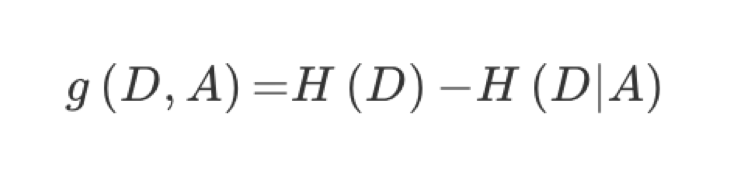公式的详细解释：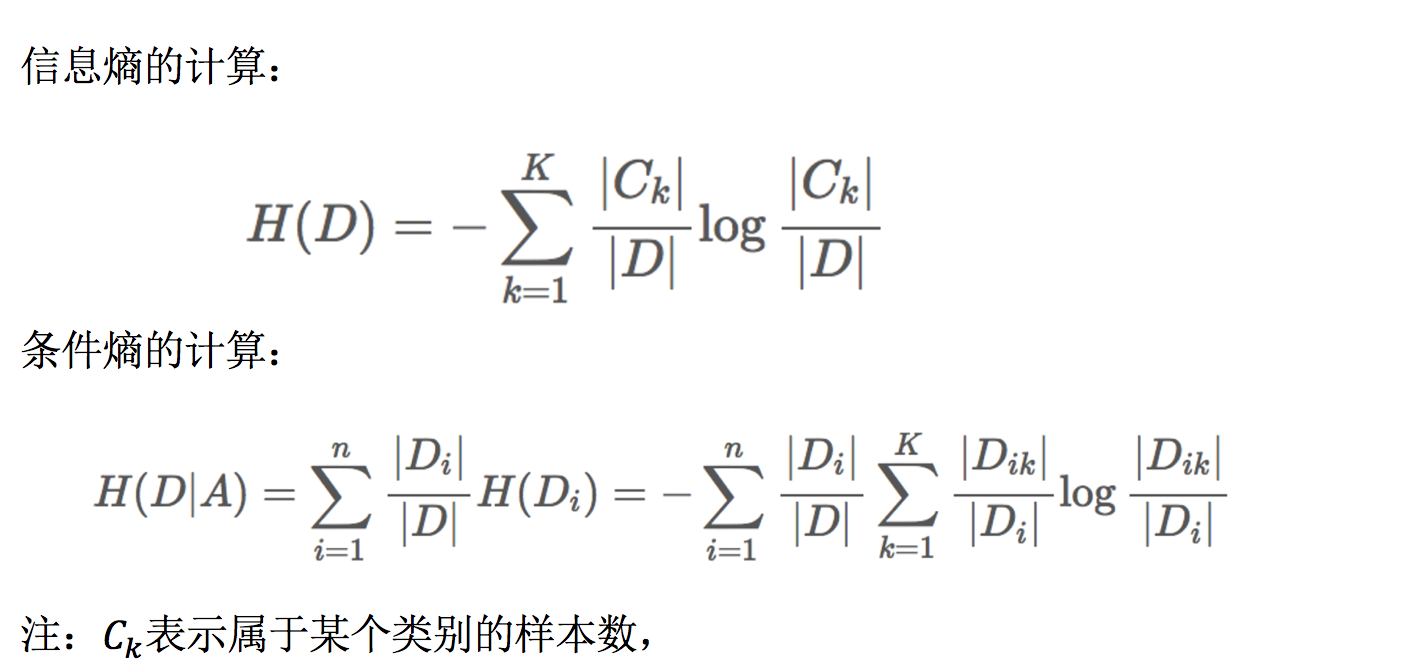注：信息增益表示得知特征X的信息而息的不确定性减少的程度使得类Y的信息熵减少的程度

6.2.3.2 贷款特征重要计算我们以年龄特征来计算：
1、g(D, 年龄) = H(D) -H(D|年龄) = 0.971-[5/15H(青年)+5/15H(中年)+5/15H(老年]

2、H(D) = -(6/15log(6/15)+9/15log(9/15))=0.971

3、H(青年) = -(3/5log(3/5) +2/5log(2/5))
H(中年)=-(3/5log(3/5) +2/5log(2/5))
H(老年)=-(4/5og(4/5)+1/5log(1/5))

6.2.4 决策树的三种算法实现
决策树的原理不止信息增益这一种，还有其他方法。但是原理都类似。
ID3:
信息增益 最大的准则
C4.5:
信息增益比 最大的准则
CART:
分类树: 基尼系数 最小的准则 在sklearn中可以选择划分的默认原则
优势：划分更加细致（从后面例子的树显示来理解）
6.2.5 决策树API
class sklearn.tree.DecisionTreeClassifier(criterion=’gini’, max_depth=None,random_state=None)
决策树分类器
criterion:默认是’基尼’系数，也可以选择信息增益的熵’entropy’
max_depth:树的深度大小
random_state:随机数种子
其中会有些超参数：max_depth:树的深度大小
其它超参数结合随机森林讲解
6.3 案例：泰坦尼克号乘客生存预测
泰坦尼克号数据:
在泰坦尼克号和titanic2数据帧描述泰坦尼克号上的个别乘客的生存状态。这里使用的数据集是由各种研究人员开始的。其中包括许多研究人员创建的旅客名单，由Michael A. Findlay编辑。我们提取的数据集中的特征是票的类别，存活，乘坐班，年龄，登陆，home.dest，房间，票，船和性别。
1、乘坐班是指乘客班（1，2，3），是社会经济阶层的代表。
2、其中age数据存在缺失。
原始数据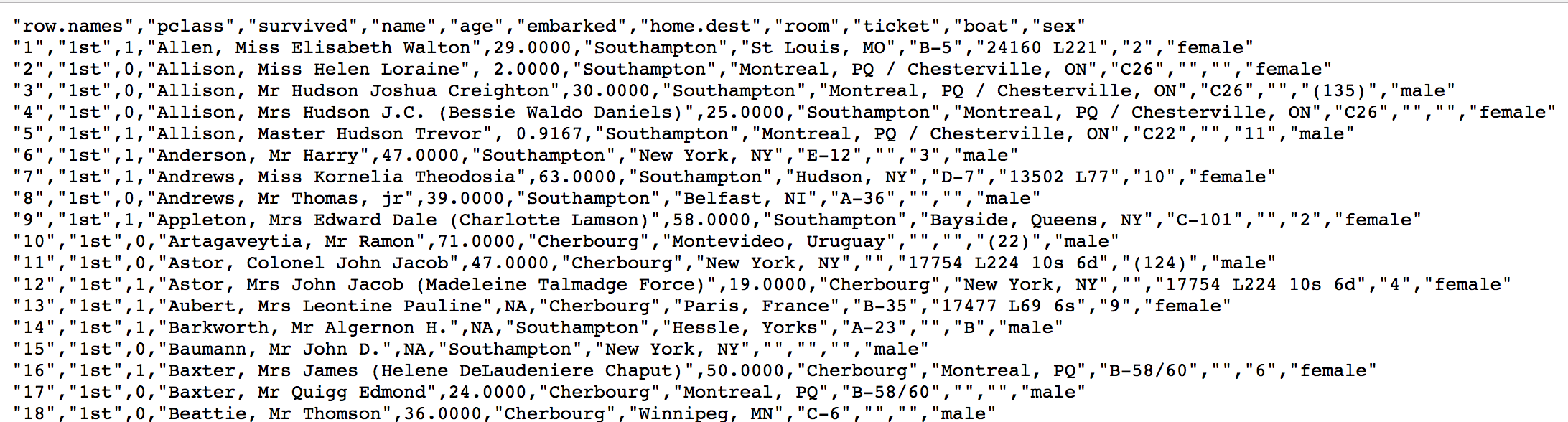6.3.1 分析
选择我们认为重要的几个特征 [‘pclass’, ‘age’, ‘sex’]
填充缺失值
特征中出现类别符号，需要进行one-hot编码处理(DictVectorizer)
x.to_dict(orient=“records”) 需要将数组特征转换成字典数据
数据集划分
决策树分类预测
6.3.2 保存树的结构到dot文件
1、sklearn.tree.export_graphviz() 该函数能够导出DOT格式
tree.export_graphviz(estimator,out_file='tree.dot’,feature_names=[‘’,’’])
2、工具:(能够将dot文件转换为pdf、png)
安装graphviz
ubuntu:sudo apt-get install graphviz Mac:brew install graphviz
3、运行命令
然后我们运行这个命令
dot -Tpng tree.dot -o tree.png
6.3.3 代码
from sklearn.feature_extraction import DictVectorizer
from sklearn.model_selection import train_test_split, GridSearchCV
from sklearn.tree import DecisionTreeClassifier, export_graphviz
import pandas as pd

def decisioncls():
"""
决策树进行泰坦尼克号乘客生存预测
:return:
"""
# 1、获取数据
taitan = pd.read_csv("http://biostat.mc.vanderbilt.edu/wiki/pub/Main/DataSets/titanic.txt")

# 2、数据的处理
x = taitan[['pclass', 'age', 'sex']]

y = taitan['survived']
#print(x, y)
# 填充,缺失值需要处理，将特征当中有类别的这些特征进行字典特征抽取
x['age'].fillna(x['age'].mean(), inplace=True)

#特征类别数据————>one-hot编码
dic = DictVectorizer(sparse=False)

# 对于x转换成字典数据x.to_dict(orient="records");将存储多个用户数据的二维数数组转换为字典
# [["lst","29","femal"],[]]---->[{"pclass": "1st", "age": 29.00, "sex": "female"}, {}]
#再进行one-hot编码
x = dic.fit_transform(x.to_dict(orient="records"))

print(dic.get_feature_names())
print(x)

# 3.分割训练集合测试集
x_train, x_test, y_train, y_test = train_test_split(x, y, test_size=0.3)

# 4.进行决策树的建立和预测
dc = DecisionTreeClassifier(max_depth=5)

dc.fit(x_train, y_train)

print("决策树预测的准确率为：", dc.score(x_test, y_test))

#导出到dot文件
export_graphviz(dc, out_file="./tree.dot",
feature_names=['age', 'pclass=1st', 'pclass=2nd', 'pclass=3rd', '女性', '男性'])

return None

if __name__=='__main__':
decisioncls()

输出结果:
['age', 'pclass=1st', 'pclass=2nd', 'pclass=3rd', 'sex=female', 'sex=male']
[[29.          1.          0.          0.          1.          0.        ]
[ 2.          1.          0.          0.          1.          0.        ]
[30.          1.          0.          0.          0.          1.        ]
...
[31.19418104  0.          0.          1.          0.          1.        ]
[31.19418104  0.          0.          1.          1.          0.        ]
[31.19418104  0.          0.          1.          0.          1.        ]]
决策树预测的准确率为： 0.8350253807106599

Process finished with exit code 0


决策树png: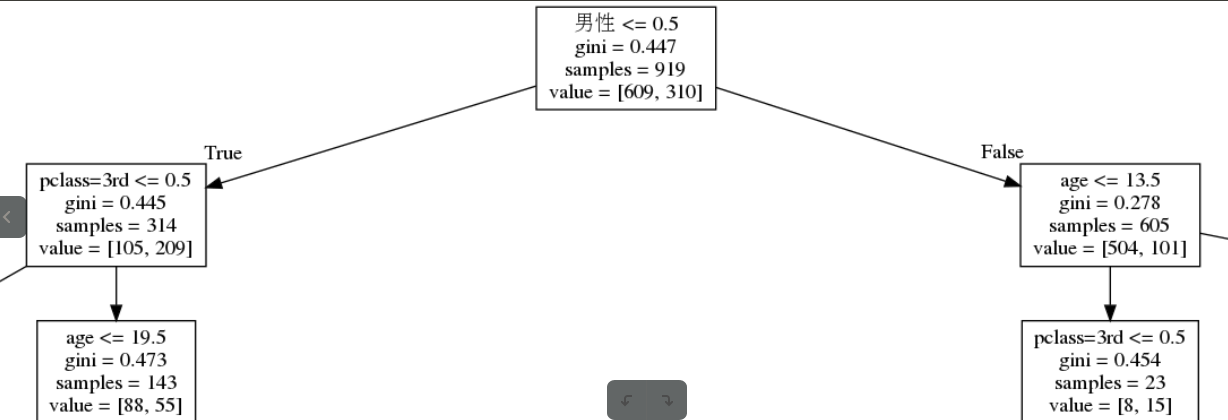6.4 总结
优点：
简单的理解和解释，树木可视化。
缺点：
决策树学习不能很好地推广数据的过于复杂的树，这被称为过拟合。
改进：
减枝cart算法(决策树API当中已经实现，随机森林参数调优有相关介绍)
注：企业用决策树算法，由于决策树很好的分析能力，在决策过程应用较多， 可以选择特征
7. 集成学习方法之随机森林
学习目标:
说名随机森林每棵决策树的建立过程
知道为什么需要随机有放回(Bootstrap)的抽样
说明随机森林的超参数
应用
泰坦尼克号乘客生存预测
7.1 什么是集成学习方法
集成学习通过建立几个模型组合的来解决单一预测问题。它的工作原理是生成多个分类器/模型，各自独立地学习和作出预测。这些预测最后结合成组合预测，因此优于任何一个单分类的做出预测。
7.2 什么是随机森林
在机器学习中，随机森林是一个包含多个决策树的分类器，并且其输出的类别是由个别树输出的类别的众数而定。例如, 如果你训练了5个树, 其中有4个树的结果是True, 1个数的结果是False, 那么最终投票结果就是True7.3 随机森林原理过程
学习算法根据下列算法而建造每棵树：
用N来表示训练用例（样本）的个数，M表示特征数目。
1、一次随机选出一个样本，重复N次， （有可能出现重复的样本）
2、随机去选出m个特征, m <<M，建立决策树
采取bootstrap抽样
7.3.1 为什么采用BootStrap抽样
为什么要随机抽样训练集？
如果不进行随机抽样，每棵树的训练集都一样，那么最终训练出的树分类结果也是完全一样的
为什么要有放回地抽样？
如果不是有放回的抽样，那么每棵树的训练样本都是不同的，都是没有交集的，这样每棵树都是“有偏的”，都是绝对“片面的”（当然这样说可能不对），也就是说每棵树训练出来都是有很大的差异的；而随机森林最后分类取决于多棵树（弱分类器）的投票表决。
7.3.2 API
class sklearn.ensemble.RandomForestClassifier(n_estimators=10, criterion=’gini’, max_depth=None, bootstrap=True, random_state=None, min_samples_split=2)
随机森林分类器
n_estimators：integer，optional（default = 10）森林里的树木数量120,200,300,500,800,1200
criteria：string，可选（default =“gini”）分割特征的测量方法
max_depth：integer或None，可选（默认=无）树的最大深度 5,8,15,25,30
max_features="auto”,每个决策树的最大特征数量
If “auto”, then max_features=sqrt(n_features).
If “sqrt”, then max_features=sqrt(n_features) (same as “auto”).
If “log2”, then max_features=log2(n_features).
If None, then max_features=n_features.
bootstrap：boolean，        optional（default = True）是否在构建树时使用放回抽样
min_samples_split:节点划分最少样本数
min_samples_leaf:叶子节点的最小样本数
超参数：n_estimator, max_depth, min_samples_split,min_samples_leaf
7.3.3 代码
from sklearn.feature_extraction import DictVectorizer
from sklearn.model_selection import train_test_split, GridSearchCV
from sklearn.ensemble import RandomForestClassifier
import pandas as pd

def randomforest():
"""
随机森林进行泰坦尼克号乘客生存预测
:return:
"""
# 1、获取数据
taitan = pd.read_csv("http://biostat.mc.vanderbilt.edu/wiki/pub/Main/DataSets/titanic.txt")

# 2、数据的处理
x = taitan[['pclass', 'age', 'sex']]
y = taitan['survived']
#print(x, y)
# 填充,缺失值需要处理，将特征当中有类别的这些特征进行字典特征抽取
x['age'].fillna(x['age'].mean(), inplace=True)

#特征类别数据————>one-hot编码
dic = DictVectorizer(sparse=False)

# 对于x转换成字典数据x.to_dict(orient="records");将存储多个用户数据的二维数数组转换为字典
# [["lst","29","femal"],[]]---->[{"pclass": "1st", "age": 29.00, "sex": "female"}, {}]
#再进行one-hot编码
x = dic.fit_transform(x.to_dict(orient="records"))

# 3.分割训练集合测试集
x_train, x_test, y_train, y_test = train_test_split(x, y, test_size=0.3)

rf = RandomForestClassifier()

# 6. 构造超参数字典
param = {"n_estimators": [120, 200, 300, 500, 1000, 1200],
"max_depth": [5, 8, 15, 25, 30],
"min_samples_split": [2, 3, 5]}

# 交叉验证
gc = GridSearchCV(rf, param_grid=param, cv=2)
gc.fit(x_train, y_train)

print("随机森林的准确率:", gc.score(x_test, y_test))
print("随机森林选择的参数:", gc.best_estimator_)

return None

if __name__=='__main__':
randomforest()

输出结果:
随机森林的准确率: 0.8121827411167513
随机森林选择的参数: RandomForestClassifier(bootstrap=True, class_weight=None, criterion='gini',
max_depth=5, max_features='auto', max_leaf_nodes=None,
min_impurity_decrease=0.0, min_impurity_split=None,
min_samples_leaf=1, min_samples_split=3,
min_weight_fraction_leaf=0.0, n_estimators=120, n_jobs=None,
oob_score=False, random_state=None, verbose=0,
warm_start=False)

Process finished with exit code 0


7.4 总结
在当前所有算法中，具有极好的准确率.
能够有效地运行在大数据集上，处理具有高维特征的输入样本，而且不需要降维.
能够评估各个特征在分类问题上的重要性.


展开全文• 用三种分类方法，分别是k最近邻、决策树朴素贝叶斯。画出数据点和决策边界，对比其区别。结果在最后图中import numpy as np from numpy import * import matplotlib.pyplot as plt from sklearn.naive_bayes ...
用三种分类方法，分别是k最近邻、决策树和朴素贝叶斯。画出数据点和决策边界，对比其区别。结果在最后的图中import numpy as np
from numpy import *
import matplotlib.pyplot as plt
from sklearn.naive_bayes import  GaussianNB
from sklearn import metrics
from sklearn.preprocessing import StandardScaler
#from sklearn.cross_validation import train_test_split
from sklearn import datasets
from matplotlib.colors import ListedColormap
from sklearn.datasets import make_moons,make_circles,make_classification
from sklearn.neighbors import KNeighborsClassifier
from sklearn.tree import DecisionTreeClassifier

h = 0.02
names = ['Nearest Neighbors','Decision Tree','Naive Bayes']
classifiers = [KNeighborsClassifier(3),DecisionTreeClassifier(max_depth=5),GaussianNB()]

#生成三种形态的数据集
X,y = make_classification(n_features=2,n_informative=2,n_redundant=0,random_state=1,n_clusters_per_class=1)
rng = np.random.RandomState(2)
X += 2 * rng.uniform(size=X.shape)
linearly_separable = (X,y)
datasets = [make_moons(noise=0.3,random_state=0),make_circles(noise=0.2,factor=0.5,random_state=1),linearly_separable]
figure = plt.figure(figsize= (18,6))
i = 1

for ds in datasets:
#处理数据，数据标准化后分为测试集和训练集 测试集占30%
X,y = ds
X = StandardScaler().fit_transform(X)
X_train = X[:int(X.shape*0.7)]
y_train = y[:int(X.shape*0.7)]
X_test = X[int(X.shape*0.7):]
y_test = y[int(X.shape*0.7):]
x_min,x_max = X[:,0].min()-0.5,X[:,0].max()+0.5
y_min, y_max = X[:, 1].min() - 0.5, X[:, 1].max() + 0.5
xx,yy = np.meshgrid(np.arange(x_min,x_max,h),np.arange(y_min,y_max))

#画出原始数据点
cm = plt.cm.RdBu
cm_bright = ListedColormap(['# FF0000','# 0000FF'])
ax = plt.subplot(len(datasets),len(classifiers)+1,i)
#填充训练集中的点
ax.scatter(X_train[:,0],X_train[:,1],c = y_train)
#填充测试集中的点
ax.scatter(X_test[:,0],X_test[:,1],c = y_test,alpha=0.6)
#设置x轴和y轴的范围
ax.set_xlim(xx.min(),xx.max())
ax.set_ylim(yy.min(), yy.max())
#设置x轴和y轴的刻度
ax.set_xticks(())
ax.set_yticks(())
i += 1
#画出每个模型的决策点和决策边界
for name,clf in zip(names,classifiers):
ax = plt.subplot(len(datasets),len(classifiers)+1,i)
score = clf.fit(X_train,y_train).score(X_test,y_test)

#画出决策边界，为此我们需要为网格中的每一个点预测一个颜色（类别）
Z = clf.predict_proba(np.c_[xx.ravel(),yy.ravel()])[:,1]
#把结果放进颜色图中
Z = Z.reshape(xx.shape)
ax.contourf(xx,yy,Z,cmap = cm,alpha = 0.8)
#填充训练集中的点
ax.scatter(X_train[:,0],X_train[:,1],c = y_train)
#填充测试集中的点
ax.scatter(X_test[:,0],X_test[:,1],c = y_test,alpha = 0.6)
ax.set_xlim(xx.min(), xx.max())
ax.set_ylim(yy.min(), yy.max())
# 设置x轴和y轴的刻度
ax.set_xticks(())
ax.set_yticks(())
ax.set_title(name)
#在图的右下角标记模型分数
ax.text(xx.max()- 0.3,yy.min()+0.3,('%.2f'%score).lstrip('0'),size = 15,horizontalalignment = 'right')
i = i+1
figure.subplots_adjust(left = 0.02,right = 0.98)
plt.show()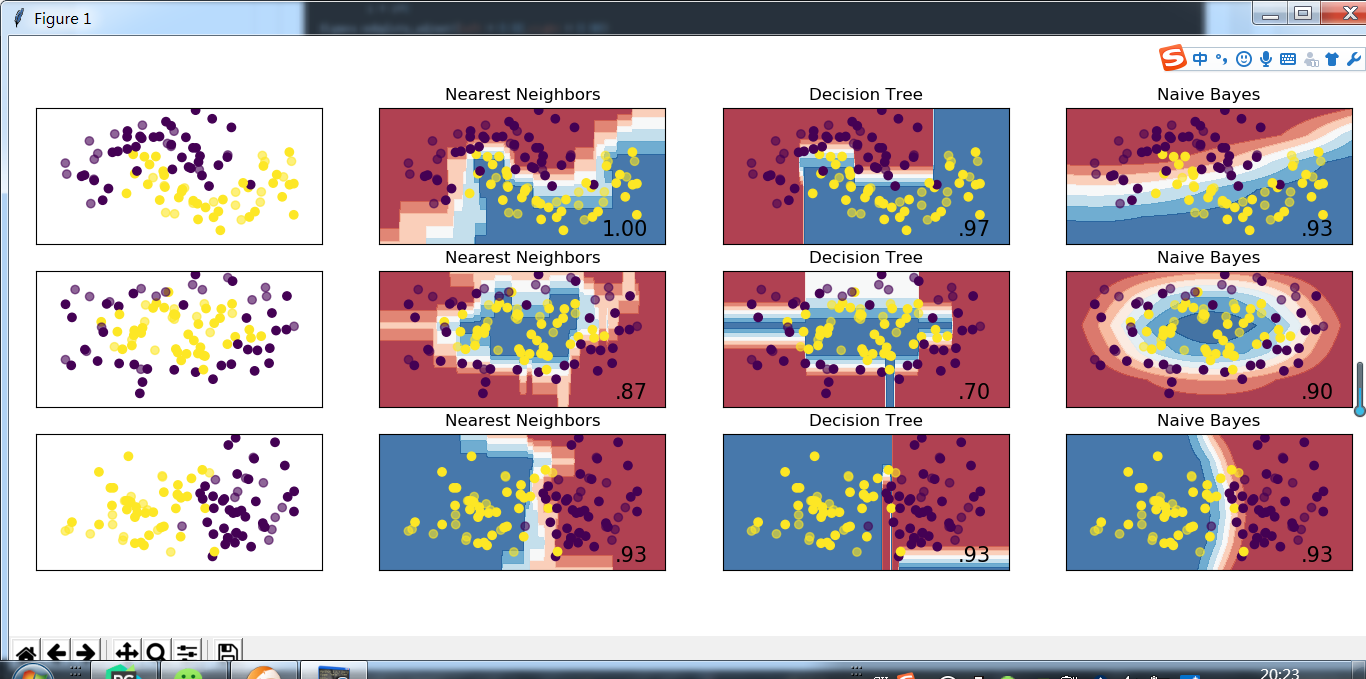展开全文k最近邻
• CARTC4.5决策树有什么区别？...为了简化决策树的规模，提高生成决策树的效率，又出现了根据GINI系数来选择测试属性的决策树算法CART。2.CART算法采用一种二分递归分割的技术，与基于信息熵的算法不同，CA...
CART和C4.5决策树有什么区别？
1.C4.5算法是在ID3算法的基础上采用信息增益率的方法选择测试属性。 ID3算法和C4.5算法虽然在对训练样本集的学习中可以尽可能多地挖掘信息，但其生成的决策树分支较大，规模较大。为了简化决策树的规模，提高生成决策树的效率，又出现了根据GINI系数来选择测试属性的决策树算法CART。2.CART算法采用一种二分递归分割的技术，与基于信息熵的算法不同，CART算法对每次样本集的划分计算GINI系数，GINI系数，GINI系数越小则划分越合理。CART算法总是将当前样本集分割为两个子样本集，使得生成的决策树的每个非叶结点都只有两个分枝。因此CART算法生成的决策树是结构简洁的二叉树。

C4.5对ID3的改进
1) 用信息增益率来选择属性，克服了用信息增益选择属性时偏向选择取值多的属性的不足；（ID3采用信息增益）
2) 在树构造过程中进行剪枝；
3) 能够完成对连续属性的离散化处理；
4) 能够对不完整数据进行处理。

CART算法
原文出自：http://www.cnblogs.com/zhangchaoyang
分类回归树(CART,Classification And Regression Tree)也属于一种决策树，上回文我们介绍了基于ID3算法的决策树。作为上篇，这里只介绍CART是怎样用于分类的。
分类回归树是一棵二叉树，且每个非叶子节点都有两个孩子，所以对于第一棵子树其叶子节点数比非叶子节点数多1。
表1
名称
体温
表面覆盖
胎生
产蛋
能飞
水生
有腿
冬眠
类标记

人
恒温
毛发
是
否
否
否
是
否
哺乳类

巨蟒
冷血
鳞片
否
是
否
否
否
是
爬行类

鲑鱼
冷血
鳞片
否
是
否
是
否
否
鱼类

鲸
恒温
毛发
是
否
否
是
否
否
哺乳类

蛙
冷血
无
否
是
否
有时
是
是
两栖类

巨蜥
冷血
鳞片
否
是
否
否
是
否
爬行类

蝙蝠
恒温
毛发
是
否
是
否
是
否
哺乳类

猫
恒温
皮
是
否
否
否
是
否
哺乳类

豹纹鲨
冷血
鳞片
是
否
否
是
否
否
鱼类

海龟
冷血
鳞片
否
是
否
有时
是
否
爬行类

豪猪
恒温
刚毛
是
否
否
否
是
是
哺乳类

鳗
冷血
鳞片
否
是
否
是
否
否
鱼类

蝾螈
冷血
无
否
是
否
有时
是
是
两栖类

上例是属性有8个，每个属性又有多少离散的值可取。在决策树的每一个节点上我们可以按任一个属性的任一个值进行划分。比如最开始我们按：
1）表面覆盖为毛发和非毛发
2）表面覆盖为鳞片和非鳞片
3）体温为恒温和非恒温
等等产生当前节点的左右两个孩子。按哪种划分最好呢？有3个标准可以用来衡量划分的好坏：GINI指数、双化指数、有序双化指数。下面我们只讲GINI指数。
GINI指数
总体内包含的类别越杂乱，GINI指数就越大（跟熵的概念很相似）。比如体温为恒温时包含哺乳类5个、鸟类2个，则：

体温为非恒温时包含爬行类3个、鱼类3个、两栖类2个,则

所以如果按照“体温为恒温和非恒温”进行划分的话，我们得到GINI的增益（类比信息增益）：

最好的划分就是使得GINI_Gain最小的划分。
终止条件
一个节点产生左右孩子后，递归地对左右孩子进行划分即可产生分类回归树。这里的终止条件是什么？什么时候节点就可以停止分裂了？直观的情况，当节点包含的数据记录都属于同一个类别时就可以终止分裂了。这只是一个特例，更一般的情况我们计算χ2值来判断分类条件和类别的相关程度，当χ2很小时说明分类条件和类别是独立的，即按照该分类条件进行分类是没有道理的，此时节点停止分裂。注意这里的“分类条件”是指按照GINI_Gain最小原则得到的“分类条件”。
假如在构造分类回归树的第一步我们得到的“分类条件”是：体温为恒温和非恒温。此时：

哺乳类
爬行类
鱼类
鸟类
两栖类

恒温
5
0
0
2
0

非恒温
0
3
3
0
2

我在《独立性检验》中讲述了χ2的计算方法。当选定置信水平后查表可得“体温”与动物类别是否相互独立。
还有一种方式就是，如果某一分支覆盖的样本的个数如果小于一个阈值，那么也可产生叶子节点，从而终止Tree-Growth。

如何确定叶子节点的类？
前面提到Tree-Growth终止的方式有2种，对于第一种方式，叶子节点覆盖的样本都属于同一类，那么这种情况下叶子节点的类自然不必多言。对于第二种方式，叶子节点覆盖的样本未必属于同一类，直接一点的方法就是，该叶子节点所覆盖的样本哪个类占大多数，那么该叶子节点的类别就是那个占大多数的类。
剪枝
当分类回归树划分得太细时，会对噪声数据产生过拟合作用。因此我们要通过剪枝来解决。剪枝又分为前剪枝和后剪枝：前剪枝是指在构造树的过程中就知道哪些节点可以剪掉，于是干脆不对这些节点进行分裂，在N皇后问题和背包问题中用的都是前剪枝，上面的χ2方法也可以认为是一种前剪枝；后剪枝是指构造出完整的决策树之后再来考查哪些子树可以剪掉。
在分类回归树中可以使用的后剪枝方法有多种，比如：代价复杂性剪枝、最小误差剪枝、悲观误差剪枝等等。

朴素贝叶斯分类方法
源自：http://www.cnblogs.com/zhangchaoyang/articles/2586402.html
我们统计了14天的气象数据(指标包括outlook，temperature，humidity，windy)，并已知这些天气是否打球(play)。如果给出新一天的气象指标数据:sunny,cool,high,TRUE，判断一下会不会去打球。
table 1
outlook
temperature
humidity
windy
play
sunny
hot
high
FALSE
no
sunny
hot
high
TRUE
no
overcast
hot
high
FALSE
yes
rainy
mild
high
FALSE
yes
rainy
cool
normal
FALSE
yes
rainy
cool
normal
TRUE
no
overcast
cool
normal
TRUE
yes
sunny
mild
high
FALSE
no
sunny
cool
normal
FALSE
yes
rainy
mild
normal
FALSE
yes
sunny
mild
normal
TRUE
yes
overcast
mild
high
TRUE
yes
overcast
hot
normal
FALSE
yes
rainy
mild
high
TRUE
no
这个问题可以用决策树的方法来求解，当然我们今天讲的是朴素贝叶斯法。这个一”打球“还是“不打球”是个两类分类问题，实际上朴素贝叶斯可以没有任何改变地解决多类分类问题。决策树也一样，它们都是有导师的分类方法。
朴素贝叶斯模型有两个假设：所有变量对分类均是有用的，即输出依赖于所有的属性；这些变量是相互独立的，即不相关的。之所以称为“朴素”，就是因为这些假设从未被证实过。
注意上面每项属性（或称指标）的取值都是离散的，称为“标称变量”。
step1.对每项指标分别统计：在不同的取值下打球和不打球的次数。
table 2
outlook
temperature
humidity
windy
play

yes
no

yes
no

yes
no

yes
no
yes
no
sunny
2
3
hot
2
2
high
3
4
FALSE
6
2
9
5
overcast
4
0
mild
4
2
normal
6
1
TRUR
3
3

rainy
3
2
cool
3
1

step2.分别计算在给定“证据”下打球和不打球的概率。
这里我们的“证据”就是sunny,cool,high,TRUE，记为E，E1=sunny,E2=cool,E3=high,E4=TRUE。
A、B相互独立时，由：

得贝叶斯定理：

得：

又因为4个指标是相互独立的，所以

我们只需要比较P(yes|E)和P(no|E)的大小，就可以决定打不打球了。所以分母P(E)实际上是不需要计算的。
P(yes|E)*P(E)=2/9×3/9×3/9×3/9×9/14=0.0053
P(no|E)*P(E)=3/5×1/5×4/5×3/5×5/14=0.0206
所以不打球的概率更大。
转载于:https://www.cnblogs.com/snapeliu/p/4605150.html
展开全文• 朴素贝叶斯模型（naive bayes） 在讲具体的原理之前，先说说朴素贝叶斯的几个...而朴素贝叶斯和隐马尔可夫模型则属于生成式模型。关于判别式模型和生成式模型的区别这里写一下： 判别式模型由数据直接学习 P(y∣x)...
 朴素贝叶斯模型（naive bayes）
注：本博客为周志华老师《机器学习》的读书笔记，但同时也参考了李航老师的《统计学习》方法，以及其他资料（见参考文献），虽有自己的理解，但博客里的例子是基于《机器学习》的。
在讲具体的原理之前，先说说朴素贝叶斯的几个特点：1、朴素贝叶斯是一种典型的生成式模型，有监督学习可以分为两类：判别模型和生成模型，我们所熟悉的神经网络，支持向量机和logistic regression，决策树等都是判别模型。而朴素贝叶斯和隐马尔可夫模型则属于生成式模型。关于判别式模型和生成式模型的区别这里写一下：
判别式模型由数据直接学习 $P(y|x)$来预测$y$，而生成式模型则是学习每个类别对应的分布$P(x|y)$和$P(y)$（也就是联合概率$P(x,y)$，$P(x,y)=P(x|y)P(y)$）。用个通俗易懂的例子来解释下（摘自ng：Generative Learning algorithms），假如我们的任务是个二分类任务，要算法根据训练数据判断出是大象（label=0）还是狗（label=1），判别式模式直接对$P(y|x)$建模，学习的是大象和狗之间的区别（Machine Learning: Generative and Discriminative Models，p10）。而生成式模型则分别对大象建模 $P(x|y=0)$和对狗建模$P(x|y=1）$，也就是用一个模型来刻画大象，再用另一个模型来刻画狗。来了一个新样本后，它去分别和大象和狗的模型进行匹配，看看更像哪一个，则把新样本分为哪一类。也就是说，生成式模型实际上是建立一个多模型（有多少类就建多少个模型），而判别式模型只有一个模型。
这两种方法各有优缺点，判别模型可以简化学习问题，由于直接面对预测，往往学习的准确率更高（判别式模型性能通常较好，这一点在Ng和Jordan的论文的论文中也说明了，论文：On Discriminative vs. Generative classifiers: A comparison of logistic regression and naive Bayes ）这一篇文章是一篇很经典的阐述判别式模型和生成式模型的论文），而生成式模型的学习收敛速度更快，当样本数量增加的时候，学到的模型可以更快的收敛于真实模型。而且可以学习有因变量的任务，比如隐马尔科夫模型。
Note：国内的书李航老师的《统计学习方法》和周志华老师的《机器学习》对生成模型的定义，皆为：先对联合概率$P(x,y)$建模，然后由此求得$P(y|x)$。虽然本质上和Ng讲的是一样的，但感觉ng的note更好理解，强烈建议大家看一下，note：Generative Learning algorithms，毕竟关于判别模型和生成模型，ng和他的导师Jordan在02年发表了一篇经典论文。
再回到朴素贝叶斯模型的话题上，我们先来看看贝叶斯决策理论，来一点点引出朴素贝叶斯模型，根据贝叶斯公式有：
$P(y|x)=\frac{p(x|y)\cdot p(y)}{p(x)} \tag{1}$
其实贝叶斯公式用更加朴素的语言就是：
$后验 = \frac{似然\times先验}{证据}\tag{2}$
其中，$P(y)$是先验概率，$P(x|y)$ 是样本$x$相对于类别$y$的条件概率（也称似然），$P(x)$是一个与$y$无关的归一化因子，因此它对所有类别来说都是相同的，其实就是个常数，因此：
$P(y|x) \propto p(x|y)\cdot p(y)\tag{3}$
设样本$x$有$n$个特征为：$x_1,x_2,x_3,...,x_n$，则上式3为：
$P(y \mid x_1, \dots, x_n) \propto P(y) P(x_1, \dots x_n \mid y)\tag{4}$
而根据条件概率的乘法公式，又有：
$P(x_1, \dots x_n \mid y) = P(x_1|y)\cdot P(x_2|x_1,y)\cdot P(x_3|x_1,x_2,y)\cdot \cdot \cdot P(x_n|x_1,x_2,....,x_{n-1},y)\tag{5}$
到这一步，发现没法做了，因为复杂度太高了，后面的多元联合概率没法算。即使对于二元分类，我们的特征$x_i$是二值的，那么复杂度也有$2^n$这种指数级的。因此朴素贝叶斯给出了一个神假设：假设特征之间是独立的。所谓 “朴素” 二字就体现在这个地方，但是根据我们的常识也知道，样本的特征之间几乎不太可能是相互独立的，因此朴素贝叶斯效果肯定不好，但结果却恰恰相反，无数的实验证明朴素贝叶斯对于文本分类任务效果都很好。
有了特征之间是相互独立的这个假设后，则上面的公式变为：
$\begin{array}{lcl} P(y|x_1,x_2,...,x_n)&=&\frac{P(y)p(x_1,x_2,...,x_n|y)}{P(x_1,x_2,...,x_n)}\\\\ &\propto& P(y)\cdot P(x_1,x_2,...,x_n|y)\\\\ &=&P(y)\cdot P(x_1|y)\cdot P(x_2|x_1,y)\cdot P(x_3|x_1,x_2,y)\cdot \cdot \cdot P(x_n|x_1,x_2,....,x_{n-1},y)\\\\ &=&P(y)\cdot P(x_1|y)\cdot P(x_2|y)\cdot P(x_3|y)\cdot \cdot \cdot P(x_n|y)\\\\ &=&P(y)\cdot \prod_{i=1}^{n}P(x_i|y) \end{array}\tag{6}$
我们只需最大化后验概率，即：
$\hat{y} = \arg\max_y P(y) \prod_{i=1}^{n} P(x_i \mid y) \tag{7}$
再回想下上面说的，生成式模型会给每个类别都建立一个模型（分布），然后新样本过来后计算与哪个分布更匹配，就划归到哪一类中，而朴素贝叶斯算法用一句话简短直白的说就是：算出新样本在每个类别的后验概率，然后看哪个最大，就把新样本分到哪个类中。还有一点需要注意的是，朴素贝叶斯并不需要像神经网络，logistic 回归和线性回归这样先根据训练数据去学习参数，然后预测新样本。朴素贝叶斯是直接那新样本去数据集（训练集）去算后验概率，然后分类，这一点与knn很相似，都是不需要训练的。
我们接下来看看公式（7）中，$P(y)$和$P(x_i|y)$怎么求，根据大数定律，若样本量充足，则有：
$P(y_c) = \frac{|D_c|}{|D|}\tag{8}$
$P(x_i|y) = \frac{|D_{c,x_i}|}{|D_c|}\tag{9}$
其中，$D_c$表示训练集$D$中第$c$类样本的数量，$|D_{c,x_i}|$表示$D_c$中第$i$个属性上取值为$x_i$的样本个数。
那么问题来了，对于分类任务而言，类别肯定是离散的，那么特征取值可不一定啊，有可能是离散的也有可能是连续的。上面的公式（9）只适用于特征值为离散的情况，那么对于特征值是连续的，该怎么做呢？这也是生成式模型的特点，需要假设分布，在朴素贝叶斯中，对于连续值，假设其符合高斯分布，即: $P(x_i|y) \sim N(\mu_{c,i},\sigma^2_{c,i})$
$P(x_i|y) = \frac{1}{\sqrt{2 \pi}\sigma_{c,i}}exp(-\frac{(x_i - \mu_{c,i})^2}{2\sigma^2_{c,i}}) \tag{10}$
其中，$\mu_{c,i},\sigma_{c,i}^2$为分别第$c$类样本在第$i$个属性上的均值和方差。
这其实就是朴素贝叶斯的原理了，基本就是这些内容，是不是真的很简单直白。在实际使用中（实现），往往还需要做个平滑，防止概率出现0的情况，这个我们下面会讲，这里先提一下。我们先来举个例子，看看在朴素贝叶斯到底是怎么实现分类的。这里的例子以及数据集，均来自周志华老师的《机器学习》书，数据集：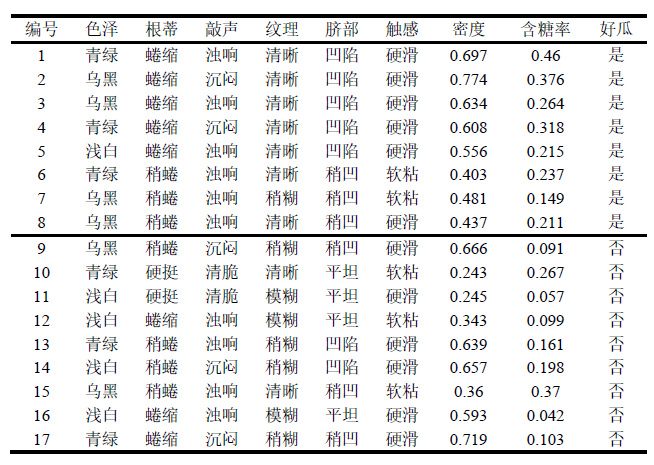我们取第一条样本当做测试样本，即：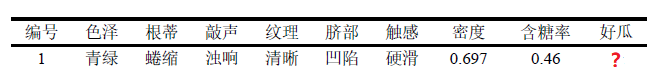下面是计算过程：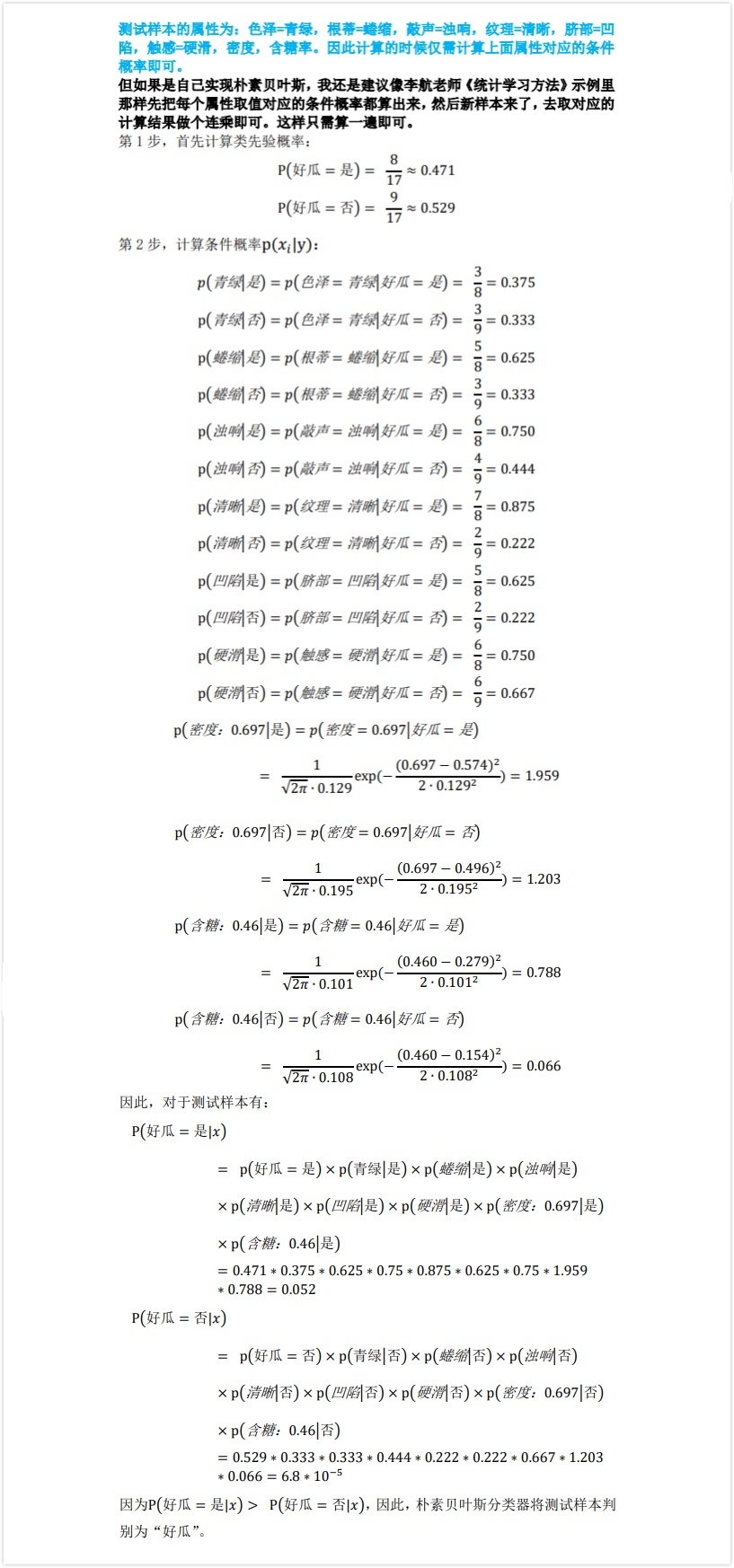目前看似一切很完美，但是其实暗藏一个bug，上面计算每个类别的概率是个连乘，连乘就会有问题，如果其中一项为0，则结果直接为0。比如，我们有个测试样本的特征为：敲声=清脆，基于上面的数据集，能够发现
$P(清脆|是) = P(敲声=清脆|好瓜=是) = 0/8 = 0$
因此，**即使其它所有特征都很符合好瓜，但是也会被判定为坏瓜。这显然是不合理的。**因此，就要用到上面提到的平滑技术，对这个概率值进行平滑，这里用拉普拉斯平滑，贝叶斯里的拉普拉斯推导就不写了，有兴趣的可以看下李航老师的《统计学习方法》或者ng的那个note，有个简短的推导。这里直接给出计算公式：
$\widehat{P}(c) = \frac{|D_c| + 1}{|D| + N} \tag{11}$
$\widehat{P}(x_i|c) = \frac{|D_{c,x_i}| + 1}{|D_c| + N_i} \tag{12}$
其中，$N$表示训练集中可能的类别数，于二分类而言，$N=2$。$N_i$表示第$i$个属性可能的取值数。
因此，在上面的例子中，加入拉普拉斯平滑后，可修正为：
$\widehat{P}(好瓜=是) = \frac{8+1}{17+2}=0.474，\widehat{P}(好瓜=否) = \frac{9+1}{17+2}=0.526 \tag{13}$
同样，
$\widehat{P}(青绿|是) = \widehat{P}(色泽=青绿|好瓜=是) \frac{3+1}{8+3}=0.364 \\ \widehat{P}(青绿|否) = \widehat{P}(色泽=青绿|好瓜=否) \frac{3+1}{9+3}=0.333$
后面的这里就不算了，感兴趣的可自行算下。显然拉普拉斯平滑解决了上文中提到的概率为0问题，而且当数据集足够大时，样本数足够多时，拉普拉斯平滑也不会对概率产生影响，几乎可以忽略不计。

参考文献
:  周志华 著. 机器学习, 北京: 清华大学出版社
:  李航 著. 统计学习方法, 北京: 清华大学出版社
: Andrew ng. CS229 Lecture notes 《Generative Learning algorithms》
: Sargur N. Srihari. Machine Learning: Generative and Discriminative Models

`
展开全文生成模型
• 1、朴素贝叶斯分类算法 和 KNN分类算法和决策树分类算法 在最终结果上面的区别是什么？ 确定分类结果：预测出实例的确定的分类结果，但是，有时候分类器会产生错误结果：KNN分类算法和决策树分类算法 最优猜测：给...
• LR、SVM、决策树等判别式模型直接生成一个表示 P(Y|X) 或者 Y=f(X) 判别函数。而生成式模型，例如朴素贝叶斯（NB），隐马尔可夫链（HMM），都是先计算联合概率分布 P(Y,X)，然后通过贝叶斯公式转化为 P(Y|X)。 ...算法 机器学习 人工智能 python
• LRSVM联系： 都是监督分类算法 都是线性分类方法 (不考虑核函数时） ...SVMLR，KNN，决策树都是判别模型，而朴素贝叶斯，隐马尔可夫模型是生成模型。  LRSVM不同 1、损失函数不同 LR是...
• 机器学习深度学习的区别 1.特征提取方面： 机器学习：手动进行特征工程 深度学习：算法自动筛选提取 ...机器学习：朴素贝叶斯决策树等 深度学习：神经网络 4.应用场景 图像，语音，文本 其它的都可...
• LR、SVM、决策树等判别式模型直接生成一个表示P(Y|X)或者Y=f(X)判别函数。而生成式模型，例如朴素贝叶斯（NB），隐马尔可夫链（HMM），都是先计算联合概率分布P(Y,X)，然后通过贝叶斯公式转化为P(Y|X...机器学习
• 2.3.朴素贝叶斯和决策树 2.4.ctr预估和协同过滤 2.5.典型自然语言和图像识别 3.数据分析和机器学习的区别 最大的区别就是数据分析是分析过去的情况，机器学习是预测未来的情况 4.机器学习的常见算法分类 ...
• 简单解释： 分类回归的区别在于输出变量的类型。 定量输出称为回归，或者说是连续变量预测；...分类算法有感知机、K近邻、朴素贝叶斯决策树、逻辑回归与最大熵模型、支持向量机、提升方法。 原始的感知机、支持机器学习 分类算法 逻辑回归 深度学习
• 决策树和随机森林的区别是什么？ 朴素贝叶斯为什么“朴素naive”？ kmeans初始点除了随机选取之外的方法 LR明明是分类模型为什么叫回归 梯度下降如何并行化 梯度下降如何并行化 LR中的L1/L2正则项是啥 简述决策树...深度学习 神经网络 数据挖掘 机器学习
• 机器学习概述（2）–生成模型判别模型的区别 k近邻 k-近邻算法（k-nearest neighbor） logistic回归 logistic回归–基本理论（1） logistic回归–最大熵模型（2） Logistic回归模型的实现（3） 决策树 决策树...机器学习
• 生成模型（Generative model）判别模型（Discriminative model） 典型的生成模型判别模型分别有哪一些 生成模型 判别模型 ...1. HMM 2. 朴素贝叶斯 3....生成模型判别模型的区别 生成方法...
• 一、机器学习深度学习的区别 机器学习先需要人工特征提取，再输入分类器；...代表算法：机器学习–朴素贝叶斯决策树等；深度学习–神经网络’ 二、Tensorflow的结构 1）案例： import tensorflow a...
• 一、数据挖掘任务分类1、预测性描述性主要区别在于是否有目标变量2、预测性包括分类回归：（1）分类：输出变量为离散型，常见算法包括（朴素贝叶斯决策树、逻辑回归、KNN、SVM、神经网络、随机森林。...
• 前者是在标注好了训练集上训练学习器，并用训练好学习器去对新样本进行预测，朴素贝叶斯决策树、神经网络等都属于这类机器学习算法。后者是在未标注数据集上根据数据本身分布情况来对数据进行分类，各种...
• KNN： 逻辑回归：线性回归有什么不同 ...朴素贝叶斯朴素贝叶斯的原理公式 Apriori：支持度置信度还有提升度是什么意思，FP-Growth的区别 FP-Growth：原理 时间序列ARMA：pq如何确定 ...机器学习
• 交叉熵公式 逻辑回归（二分类）LR损失函数 M为样本个数,为模型对样本i...单一分类方法主要包括：LR逻辑回归，SVM支持向量机，DT决策树、NB朴素贝叶斯、NN人工神经网络、K-近邻；集成学习算法：基于BaggingBoo逻辑回归 机器学习
• ## 机器学习复习

千次阅读 2018-11-21 21:48:24
文章目录SVM**balance的方法**逻辑回归SVM的联系区别朴素贝叶斯逻辑回归线性回归KNNKD树决策树随机森林GBDTShrinkage最小二乘法随机森林的区别EMBaggingBoosting凸集凸函数daBoost分类回归的区别生成模型...
• ## 算法工程师面试

千次阅读 2018-12-25 21:46:59
决策树的ID3，C4.5，CART等，决策树的split原理剪枝策略 神经网络如何工作 SVM的原理及公式推导 朴素贝叶斯的公式 Bagging Boosting的区别 GBDT的参数怎么调 聚类过程 偏差方差是什么，高偏差高方差说明了...
• 机器学习理论： 1. 写出全概率公式&...6. 决策树和随机森林的区别是什么？ 7. 朴素贝叶斯 为什么 “朴素 naive” 8. Kmeans 初始点除了随机选取之外的方法？ 9. LR 明明是分类模型为什么叫回归？ 10. 梯度.
• 机器学习理论类： 1. 写出全概率公式&...6. 决策树和随机森林的区别是什么？ 7. 朴素贝叶斯为什么“朴素naive”？ 8. kmeans初始点除了随机选取之外的方法 9. LR明明是分类模型为什么叫回归...
• 目录 1. 写出全概率公式&...6. 决策树和随机森林的区别是什么？ 7. 朴素贝叶斯为什么“朴素naive”？ 8. kmeans初始点除了随机选取之外的方法 9. LR明明是分类模型为什么叫回归 10. 梯度下降如何并行化 .
• 2.17.1 决策树的基本原理理 2.17.2 决策树的三要素? 2.17.3 决策树学习基本算法 2.17.4 决策树算法优缺点 2.17.5熵的概念以及理理解 2.17.6 信息增益的理理解 2.17.7 剪枝处理理的作⽤用及策略略? 2.18 ⽀支持向量量...
• 1.常用算法 决策树 随机森林算法 逻辑回归 SVM 朴素贝叶斯 Knn算法 K-means算法 Adaboost 算法 神经网络 ...2.聚类分类的区别 分类：比如有一堆数据，你已经事先对他们做好了分机器学习
• 文章目录一、机器学习生成模式判别模式的区别：感知机：K-means：k近邻：朴素贝叶斯：极大似然估计：逻辑回归(LR)：L1L2的区别FP(FP-growth)关联算法支持向量机(SVM)：决策树：bagging随机森林：boosting梯度...算法
• ## 划重点

千次阅读 2017-02-22 19:16:13
朴素贝叶斯推倒 逻辑回归 （重点）推倒  频率学派贝叶斯学派 生成方法判别方法 ID3/C4.5/CART 决策树 熵、互信息、KL散度 过拟合、正则化 偏差方差 感知机推倒 k-means聚类推倒 KKT条